• 点上方人工智能算法与Python大数据获取更多干货在右上方···设为星标★，第一时间获取资源仅做学术分享，如有侵权，联系删除转载于 ：机器之心算法完美是重要的，但更重要的是成功部署，...

点上方人工智能算法与Python大数据获取更多干货

在右上方 ··· 设为星标 ★，第一时间获取资源

仅做学术分享，如有侵权，联系删除

转载于 ：机器之心

算法完美是重要的，但更重要的是成功部署，这篇文章能够帮助你了解有关代码内存占用的一切。

在进行机器学习任务时，你需要学会使用代码快速检查模型的内存占用量。原因很简单，硬件资源是有限的，单个机器学习模块不应该占用系统的所有内存，这一点在边缘计算场景中尤其重要。

比如，你写了一个很棒的机器学习程序，或者搭建了一个不错的神经网络模型，然后想在某些 Web 服务或 REST API 上部署模型。或者你是基于工厂传感器的数据流开发了模型，计划将其部署在其中一台工业计算机上。

这时，你的模型可能是硬件上运行的几百个模型之一，所以你必须对内存占用峰值有所了解。否则多个模型同时达到了内存占用峰值，系统可能会崩溃。

因此，搞清楚代码运行时的内存配置文件（动态数量）非常重要。这与模型的大小和压缩均无关，可能是你事先已经将其保存在磁盘上的特殊对象，例如 Scikit-learn Joblib dump、Python Pickle dump，TensorFlow HFD5 等。

Scalene：简洁的内存 / CPU/GPU 分析器

首先要讨论的是 Scalene，它是一个 Python 的高性能 CPU 和内存分析器，由马萨诸塞大学研发。其 GitHub 页面是这样介绍的：「 Scalene 是适用于 Python 的高性能 CPU、GPU 和内存分析器，它可以执行许多其他 Python 分析器无法做到的事情，提供详细信息比其他分析器快几个数量级。」

安装

它是一个 Python 包，所以按照通常方法安装：

pip install scalene

这样适用于 Linux OS，作者没有在 Windows 10 上进行测试。

在 CLI 或 Jupyter Notebook 内部使用

Scalene 的使用非常简单：

scalene <yourapp.py>

也可以使用魔术命令在 Jupyter notebook 中使用它：

输出示例

下面是一个输出示例。稍后将对此进行更深入的研究。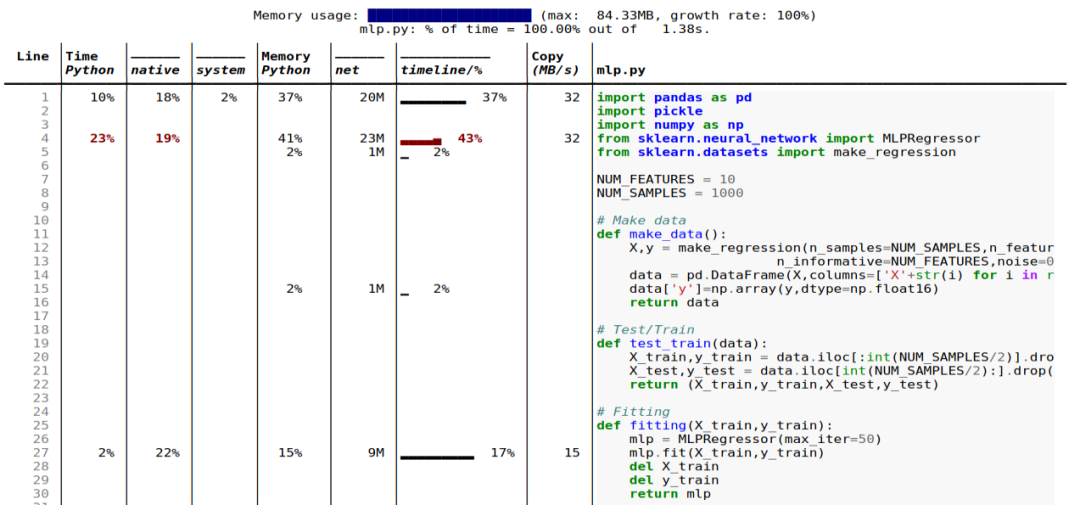这些是 Scalene 一些很酷的功能：

• 行和函数：报告有关整个函数和每个独立代码行的信息；

• 线程：支持 Python 线程；

• 多进程处理：支持使用 multiprocessing 库；

• Python 与 C 的时间：Scalene 用在 Python 与本机代码（例如库）上的时间；

• 系统时间：区分系统时间（例如，休眠或执行 I / O 操作）；

• GPU：报告在英伟达 GPU 上使用的时间（如果有）；

• 复制量：报告每秒要复制的数据量；

• 泄漏检测：自动查明可能造成内存泄漏的线路。

ML 代码具体示例

接下来看一下 Scalene 用于内存配置标准机器学习代码的工作。对三个模型使用 Scikit-learn 库，并利用其综合数据生成功能来创建数据集。

对比的是两种不同类型的 ML 模型：

• 多元线性回归模型；

• 具有相同数据集的深度神经网络模型。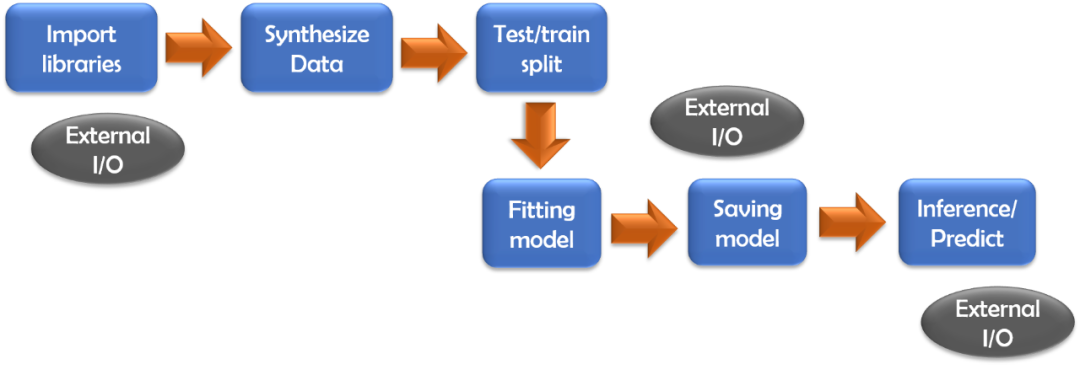线性回归模型

使用标准导入和 NUM_FEATURES 、 NUM_SMPLES 两个变量进行一些实验。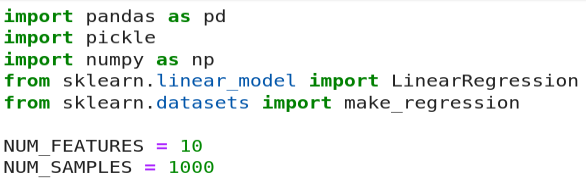这里没有展示数据生成和模型拟合代码，它们是非常标准的。作者将拟合的模型另存为 pickled dump，并将其与测试 CSV 文件一起加载以进行推断。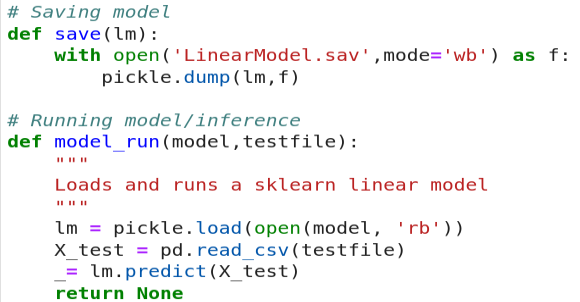为了清晰起见，将所有内容置于 Scalene 执行和报告环境下循环运行。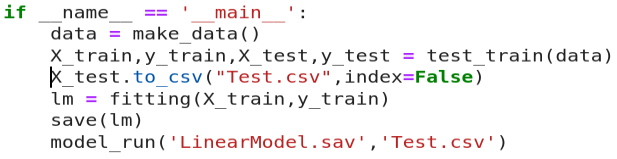当运行命令时：

\$ scalene linearmodel.py --html >> linearmodel-scalene.html

将这些结果作为输出。注意，此处使用了 --html 标志并将输出通过管道传输到 HTML 文件，以便于报告。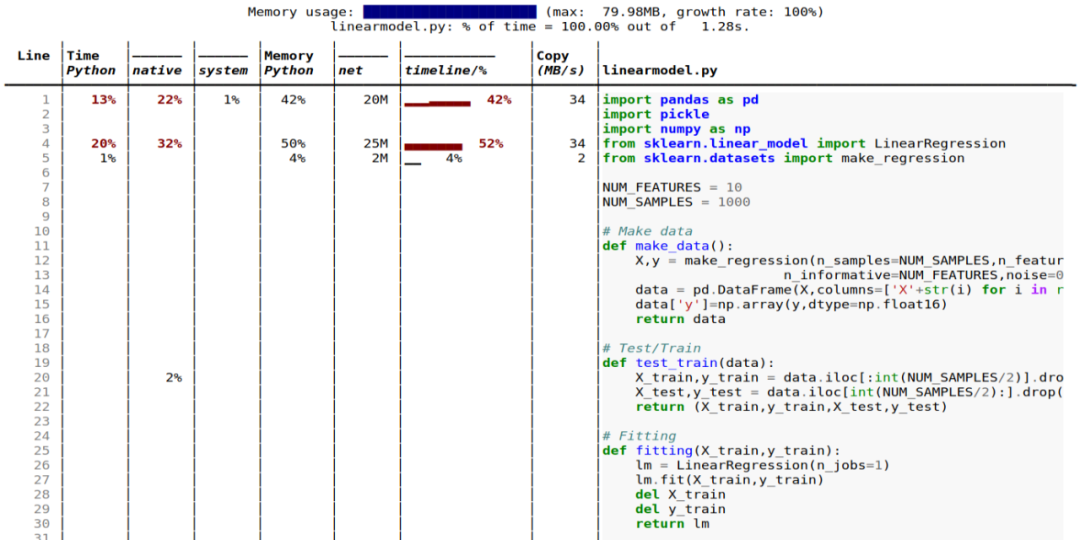令人惊讶的是，内存占用几乎完全由外部 I / O（例如 Pandas 和 Scikit-learn estimator 加载）控制，少量会将测试数据写到磁盘上的 CSV 文件中。实际的 ML 建模、Numpy、Pandas 操作和推理，根本不会影响内存。

我们可以缩放数据集大小（行数）和模型复杂度（特征数），并运行相同的内存配置文件以记录各种操作在内存消耗方面的表现。结果显示在这里。

此处，X 轴代表特征 / 数据点集。注意该图描绘的是百分比，而不是绝对值，展示了各种类型操作的相对重要性。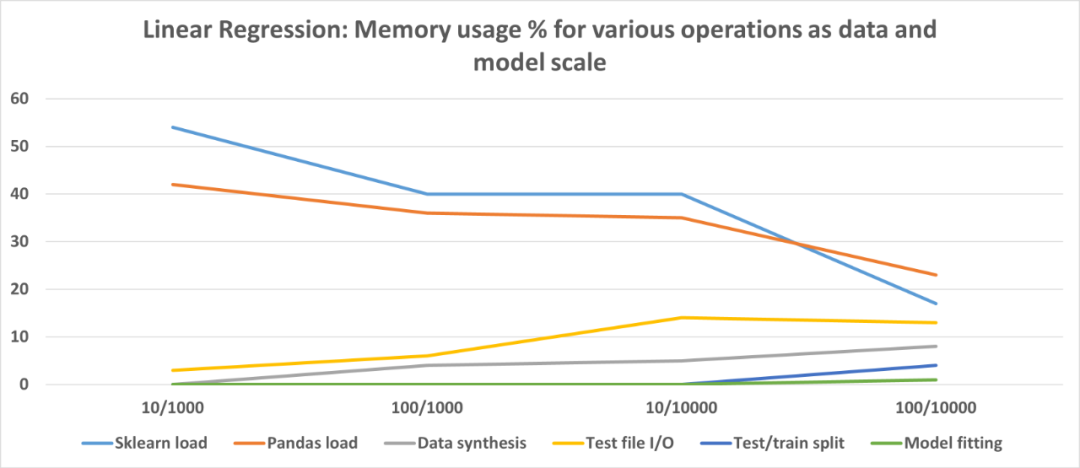从这些实验中得出的结论是，Scikit-learn 线性回归估计非常高效，并且不会为实际模型拟合或推理消耗大量内存。

但就代码而言，它确实有固定的内存占用，并在加载时会消耗大量内存。不过随着数据大小和模型复杂性的增加，整个代码占用百分比会下降。如果使用这样的模型，则可能需要关注数据文件 I / O，优化代码以获得更好的内存性能。

深度神经网络如何？

如果我们使用 2 个隐藏层的神经网络（每个隐藏层有 50 个神经元）运行类似的实验，那么结果如下所示。

代码地址：https://github.com/tirthajyoti/Machine-Learning-with-Python/blob/master/Memory-profiling/Scalene/mlp.py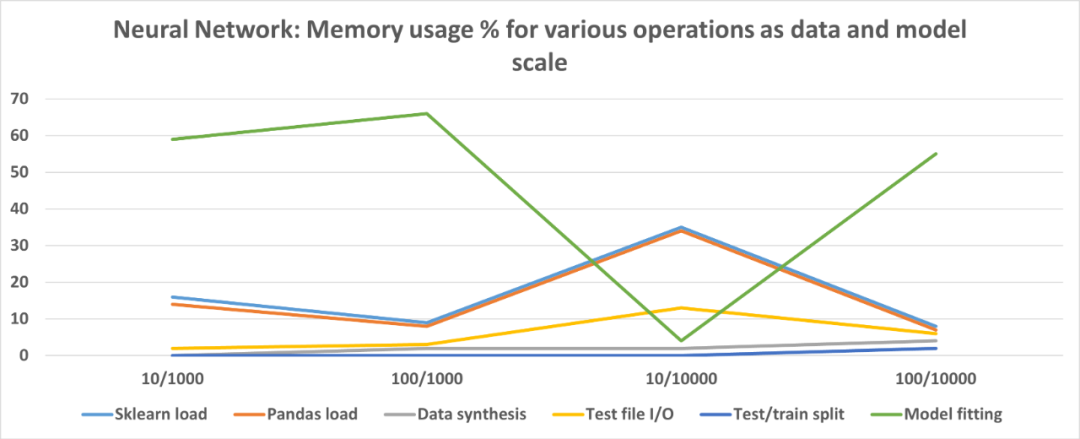与线性回归模型不同，神经网络模型在训练 / 拟合步骤中消耗大量内存。但是，由于特征少且数据量大，拟合占用的内存较少。此外，还可以尝试各种体系结构和超参数，并记录内存使用情况，达到合适的设置。

复现说明

如果你使用相同的代码复现实验，结果可能会因硬件、磁盘 / CPU / GPU / 内存类型的不同而大相径庭。

一些关键建议

• 最好在代码中编写专注于单个任务的小型函数；

• 保留一些自由变量，例如特征数和数据点，借助最少的更改来运行相同的代码，在数据 / 模型缩放时检查内存配置文件；

• 如果要将一种 ML 算法与另一种 ML 算法进行比较，请让整体代码的结构和流程尽可能相同以减少混乱。最好只更改 estimator 类并对比内存配置文件；

• 数据和模型 I / O（导入语句，磁盘上的模型持久性）在内存占用方面可能会出乎意料地占主导地位，具体取决于建模方案，优化时切勿忽略这些；

• 出于相同原因，请考虑比较来自多个实现 / 程序包的同一算法的内存配置文件（例如 Keras、PyTorch、Scikitlearn）。如果内存优化是主要目标，那么即使在功能或性能上不是最佳，也必须寻找一种占用最小内存且可以满意完成工作的实现方式；

• 如果数据 I / O 成为瓶颈，请探索更快的选项或其他存储类型，例如，用 parquet 文件和 Apache Arrow 存储替换 Pandas CSV。可以看看这篇文章：

《How fast is reading Parquet file (with Arrow) vs. CSV with Pandas?》

Scalene 能做的其他事

在本文中，仅讨论了内存分析的一小部分，目光放在了规范机器学习建模代码上。事实上 Scalene CLI 也有其他可以利用的选项：

• 仅分析 CPU 时间，不分析内存；

• 仅使用非零内存减少资源占用；

• 指定 CPU 和内存分配的最小阈值；

• 设置 CPU 采样率；

• 多线程并行，随后检查差异。

最终验证（可选）

在资源较少的情况下，你最好托管一个验证环境 / 服务器，该服务器将接受给定的建模代码（如已开发），并通过这样的内存分析器运行它以创建运行时统计信息。如果它通过内存占用空间的预定标准，则只有建模代码会被接受用于进一步部署。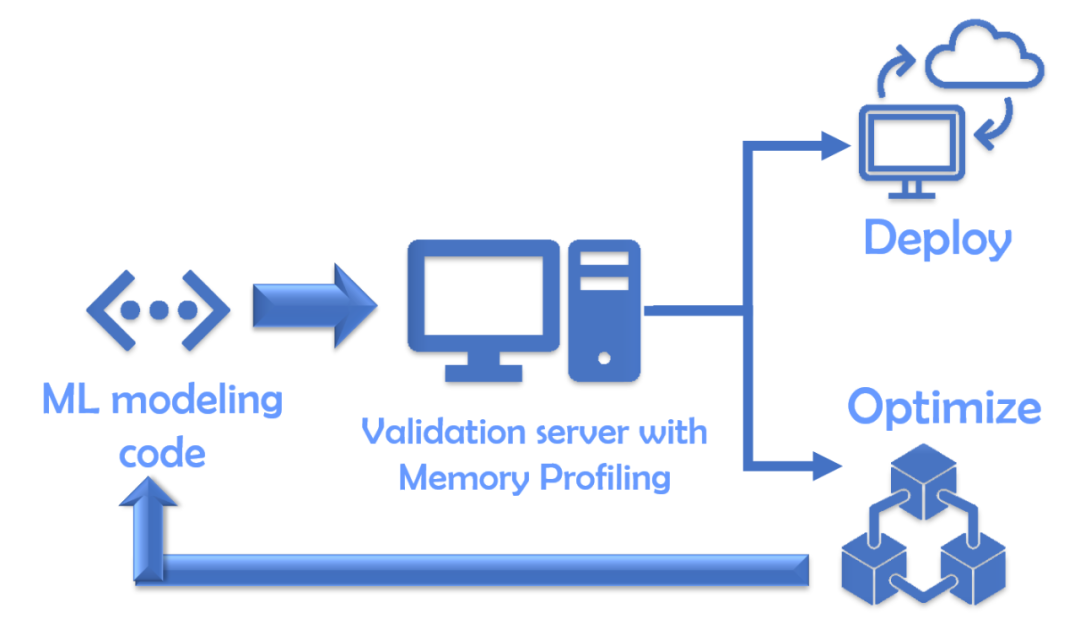总结

在本文中，我们讨论了对机器学习代码进行内存配置的重要性。我们需要使其更好地部署在服务和机器中，让平台或工程团队能够方便运用。分析内存也可以让我们找到更高效的、面向特定数据或算法的优化方式。

希望你能在使用这些工具和技术进行机器学习部署时能够获得成功。

原文链接：https://towardsdatascience.com/how-much-memory-is-your-ml-code-consuming-98df64074c8f

---------♥---------

声明：本内容来源网络，版权属于原作者

图片来源网络，不代表本公众号立场。如有侵权，联系删除

AI博士私人微信，还有少量空位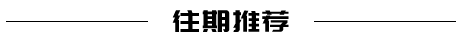一文读懂深度学习中的各种卷积

点个在看支持一下吧展开全文人工智能 算法 大数据 python 机器学习
• 9种常用的机器学习算法实现

千次阅读 2021-01-06 16:20:00
简介根据机器学习的任务或应用情况的不同，我们通常把机器学习分为三大类：1、监督学习（Supervised Learning，SL），这类算法的工作原理是使用带标签的训练数据来学习输入变...简介

根据机器学习的任务或应用情况的不同，我们通常把机器学习分为三大类：

1、监督学习（Supervised Learning，SL），这类算法的工作原理是使用带标签的训练数据来学习输入变量转化为输出变量的映射函数，换句话说就是求解方程中的。进一步地，监督学习又可细分为如下三类：

• 回归（Regression）：预测一个值，如预测降雨量、房价等，较基础的算法有：Linear Regression

• 分类（Classification）：预测一个标签，如预测“生病”或“健康”，图片上是哪种动物等，较基础的算法有：Logistic Regression、Naive Bayes、K-Nearest Neighbors（KNN）

【另】：集成（Ensembling）也可以归类为监督学习的一种，它将多个单独较弱的机器学习模型的预测结合起来，以产生更准确的预测，较基础的算法有Bagging with Random Forests、Boosting with XGBoost

2、非监督学习（Unsupervised Learning，UL），这类算法的工作原理是从无标签的训练数据中学习数据的底层结构。进一步地，非监督学习又可细分为如下三类：

• 关联（Association）：发现集合中项目同时出现的概率，如通过分析超市购物篮，发现啤酒总是和尿片一起购买（啤酒与尿片的故事），较基础的算法有：Apriori

• 聚类（Clustering）：对数据进行分组，以便组内对象比组间对象更相似，较基础的算法有：K-Means

• 降维（Dimensionality Reduction）：减少数据集的变量数量，同时保证重要的信息不被丢失。降维可以通过特征提取方法和特征选择方法来实现，特征提取是执行从高维空间到低维空间的转换，特征选择是选择原始变量的子集，较基础的算法有：PCA

3、强化学习（Reinforcement Learning，DL），让agent根据当前环境状态，通过学习能够获得最大回报的行为来决定下一步的最佳行为。

实现

以上列出的算法都是简单常用的，基于scikit-learn可以仅用几行代码就完成模型训练、预测、评估和可视化。关于算法的原理知乎上有很多精彩的回答，这里不会赘述，仅给出代码的实现与可视化。

▐  Linear Regression

它为变量分配最佳权重，以创建一条直线或一个平面或更高维的超平面，使得预测值和真实值之间的误差最小化。具体原理参考：用人话讲明白线性回归LinearRegression - 化简可得的文章 - 知乎。下面以一元线性回归为例，给出代码实现。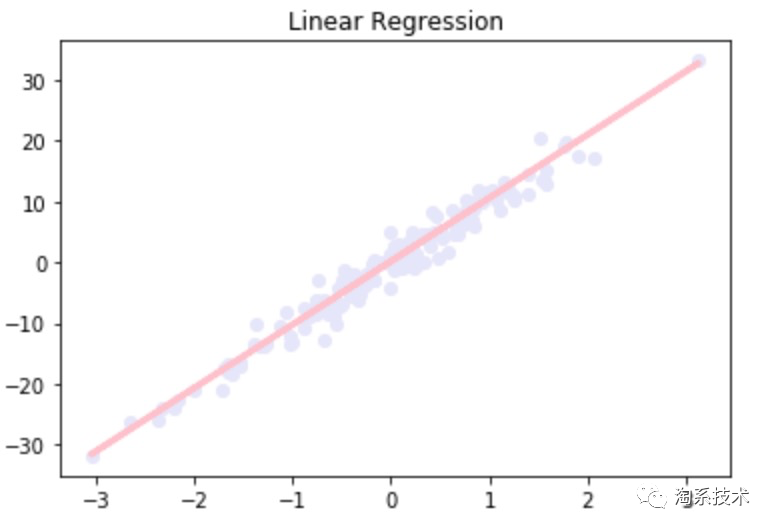import matplotlib.pyplot as pltimport numpy as npfrom sklearn import datasetsfrom sklearn.model_selection import train_test_split
# Linear Regression 一元回归from sklearn import linear_modelfrom sklearn.metrics import mean_squared_error
# 1. 准备数据lr_X_data, lr_y_data = datasets.make_regression(n_samples=500,n_features=1,n_targets=1,noise=2) # feature为1维度# 2. 构造训练与测试集lr_X_train, lr_X_test, lr_y_train, lr_y_test = train_test_split(lr_X_data, lr_y_data, test_size=0.3)# 3. 训练模型lr_model = linear_model.LinearRegression()lr_model.fit(lr_X_train, lr_y_train)# 4. 预测数据lr_y_pred = lr_model.predict(lr_X_test)# 5. 评估模型lr_mse = mean_squared_error(lr_y_test, lr_y_pred)print("mse:", lr_mse)# 6. 可视化plt.figure('Linear Regression')plt.title('Linear Regression')plt.scatter(lr_X_test, lr_y_test, color='lavender', marker='o')plt.plot(lr_X_test, lr_y_pred, color='pink', linewidth=3)plt.show()
# print info mse: 4.131366697554779

▐  Logistic Regression

虽然写着回归，但实际上是一种二分类算法。它将数据拟合到logit函数中，所以称为logit回归。简单来说就是基于一组给定的变量，用logistic function来预测这个事件的概率，给出一个介于0和1之间的输出。具体原理参考：用人话讲明白逻辑回归Logistic regression - 化简可得的文章 - 知乎，下面给出代码的实现。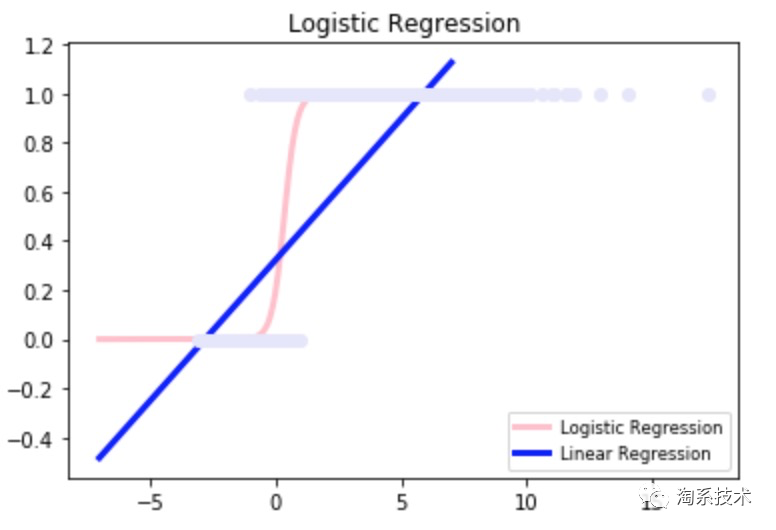import matplotlib.pyplot as plt
import numpy as np
from sklearn import datasets
from sklearn.model_selection import train_test_split

# Logistic Regression 二分类
from sklearn import linear_model

# 1. 准备数据
np.random.seed(123)
logit_X_data = np.random.normal(size=1000)
logit_y_data = (logit_X_data>0).astype(np.float)
logit_X_data[logit_X_data>0]*=5
logit_X_data+=.4*np.random.normal(size=1000)
logit_X_data=logit_X_data[:,np.newaxis]
# 2. 构造训练与测试集
logit_X_train, logit_X_test, logit_y_train, logit_y_test = train_test_split(logit_X_data, logit_y_data, test_size=0.3)
# 3. 训练模型
logit_model=linear_model.LogisticRegression(C=1e4) #classifier
logit_model.fit(logit_X_train,logit_y_train)
# 4. 预测数据
logit_y_pred = logit_model.predict(logit_X_test)
# 5. 评估模型
logit_acc = logit_model.score(logit_X_test,logit_y_pred)
print("accuracy:", logit_acc)
# 5. 可视化
logit_X_view=np.linspace(-7,7,277)
logit_X_view = logit_X_view[:,np.newaxis]
def model(x):
return 1/(1+np.exp(-x))
loss=model(logit_X_view*logit_model.coef_+logit_model.intercept_).ravel()
plt.figure('Logistic Regression')
plt.title('Logistic Regression')
plt.scatter(logit_X_train.ravel(), logit_y_train, color='lavender',zorder=17)
plt.plot(logit_X_view, loss, color='pink',linewidth=3)

lr_model=linear_model.LinearRegression()
lr_model.fit(logit_X_train,logit_y_train)
plt.plot(logit_X_view, lr_model.predict(logit_X_view), color='blue', linewidth=3)
plt.legend(('Logistic Regression','Linear Regression'),loc='lower right',fontsize='small')

# print info accuracy: 1.0

▐  Naive Bayes

朴素贝叶斯是一种基于贝叶斯定理的分类方法，它会假设一个类中的某个特征与其他特征无关。这个模型不仅非常简单，而且比许多高度复杂的分类方法表现得更好。具体原理参考：朴素贝叶斯算法原理小结 - 刘建平Pinard，下面给出代码的实现。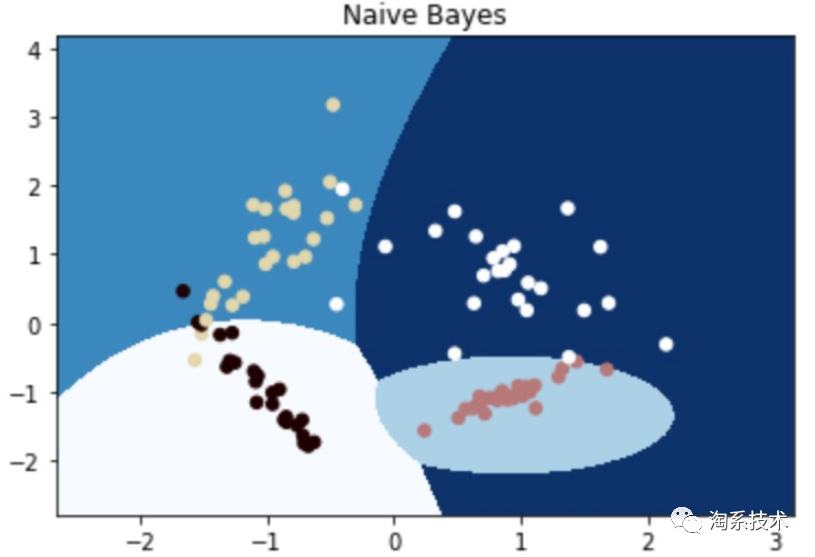import matplotlib.pyplot as plt
import numpy as np
from sklearn.model_selection import train_test_split
from sklearn.datasets import make_classification

# Naive Bayes 任务为分类, n_classes=4
import sklearn.naive_bayes as nb
# 1. 准备数据
nb_X_train, nb_y_train = make_classification(n_features=2, n_redundant=0, n_informative=2,
random_state=1, n_clusters_per_class=1, n_classes=4)
# 2. 构造训练与测试集
l, r = nb_X_train[:, 0].min() - 1, nb_X_train[:, 0].max() + 1
b, t = nb_X_train[:, 1].min() - 1, nb_X_train[:, 1].max() + 1
n = 1000
grid_x, grid_y = np.meshgrid(np.linspace(l, r, n), np.linspace(b, t, n))
nb_X_test = np.column_stack((grid_x.ravel(), grid_y.ravel()))
# 3. 训练模型
nb_model = nb.GaussianNB()
nb_model.fit(nb_X_train, nb_y_train)
# 4. 预测数据
nb_y_pred = nb_model.predict(nb_X_test)
# 5. 可视化
grid_z = nb_y_pred.reshape(grid_x.shape)
plt.figure('Naive Bayes')
plt.title('Naive Bayes')
plt.pcolormesh(grid_x, grid_y, grid_z, cmap='Blues')
plt.scatter(nb_X_train[:, 0], nb_X_train[:, 1], s=30, c=nb_y_train, cmap='pink')
plt.show()

▐  K-Nearest Neighbors

这是用于分类和回归的机器学习算法(主要用于分类)。它考虑了不同的质心，并使用欧几里得函数来比较距离。接着分析结果并将每个点分类到组中，以优化它，使其与所有最接近的点一起放置。它使用k个最近邻的多数票对数据进行分类预测。具体原来参考：K近邻法(KNN)原理小结 - 刘建平Pinard，下面给出代码的实现。

import matplotlib.pyplot as plt
import numpy as np
from sklearn.model_selection import train_test_split
from sklearn.datasets import make_classification

# Naive Bayes 任务为分类, n_classes=4
import sklearn.naive_bayes as nb
# 1. 准备数据
nb_X_train, nb_y_train = make_classification(n_features=2, n_redundant=0, n_informative=2,
random_state=1, n_clusters_per_class=1, n_classes=4)
# 2. 构造训练与测试集
l, r = nb_X_train[:, 0].min() - 1, nb_X_train[:, 0].max() + 1
b, t = nb_X_train[:, 1].min() - 1, nb_X_train[:, 1].max() + 1
n = 1000
grid_x, grid_y = np.meshgrid(np.linspace(l, r, n), np.linspace(b, t, n))
nb_X_test = np.column_stack((grid_x.ravel(), grid_y.ravel()))
# 3. 训练模型
nb_model = nb.GaussianNB()
nb_model.fit(nb_X_train, nb_y_train)
# 4. 预测数据
nb_y_pred = nb_model.predict(nb_X_test)
# 5. 可视化
grid_z = nb_y_pred.reshape(grid_x.shape)
plt.figure('Naive Bayes')
plt.title('Naive Bayes')
plt.pcolormesh(grid_x, grid_y, grid_z, cmap='Blues')
plt.scatter(nb_X_train[:, 0], nb_X_train[:, 1], s=30, c=nb_y_train, cmap='pink')
plt.show()

▐  Decision Tree

遍历树，并将重要特征与确定的条件语句进行比较。它是降到左边的子分支还是降到右边的子分支取决于结果。通常，更重要的特性更接近根，它可以处理离散变量和连续变量。具体原理参考：深入浅出理解决策树算法（一）-核心思想 - 忆臻的文章 - 知乎，下面给出代码的实现。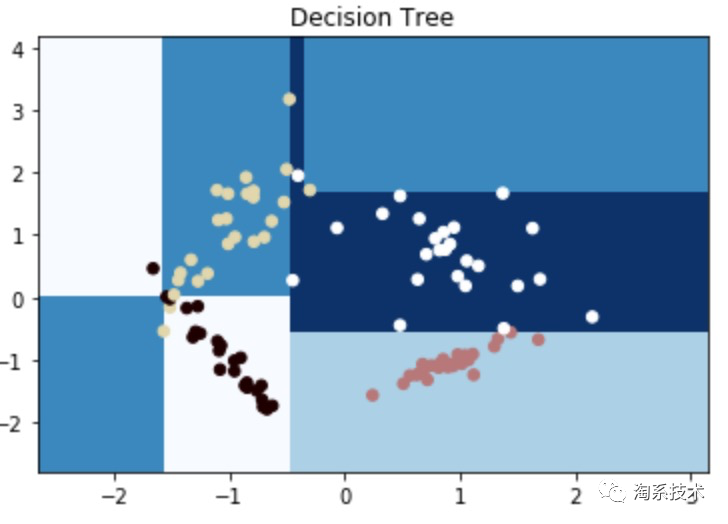import matplotlib.pyplot as plt
import numpy as np
from sklearn.model_selection import train_test_split
from sklearn.datasets import make_classification

# K-Nearest Neighbors 任务为分类, n_classes=4
from sklearn.neighbors import KNeighborsClassifier
# 1. 准备数据
knn_X_train, knn_y_train = make_classification(n_features=2, n_redundant=0, n_informative=2,
random_state=1, n_clusters_per_class=1, n_classes=4)
# 2. 构造训练与测试集
l, r = knn_X_train[:, 0].min() - 1, knn_X_train[:, 0].max() + 1
b, t = knn_X_train[:, 1].min() - 1, knn_X_train[:, 1].max() + 1
n = 1000
grid_x, grid_y = np.meshgrid(np.linspace(l, r, n), np.linspace(b, t, n))
knn_X_test = np.column_stack((grid_x.ravel(), grid_y.ravel()))
# 3. 训练模型
knn_model = KNeighborsClassifier(n_neighbors=5)
knn_model.fit(knn_X_train, knn_y_train)
# 4. 预测数据
knn_y_pred = knn_model.predict(knn_X_test)
# 5. 可视化
grid_z = knn_y_pred.reshape(grid_x.shape)
plt.figure('K-Nearest Neighbors')
plt.title('K-Nearest Neighbors')
plt.pcolormesh(grid_x, grid_y, grid_z, cmap='Blues')
plt.scatter(knn_X_train[:, 0], knn_X_train[:, 1], s=30, c=knn_y_train, cmap='pink')
plt.show()

▐  Random Forest

随机森林是决策树的集合。随机采样数据点构造树、随机采样特征子集分割，每棵树提供一个分类。得票最多的分类在森林中获胜，为数据点的最终分类。具体原来参考：独家 | 一文读懂随机森林的解释和实现 - 清华大学数据科学研究院的文章 - 知乎，下面给出代码的实现。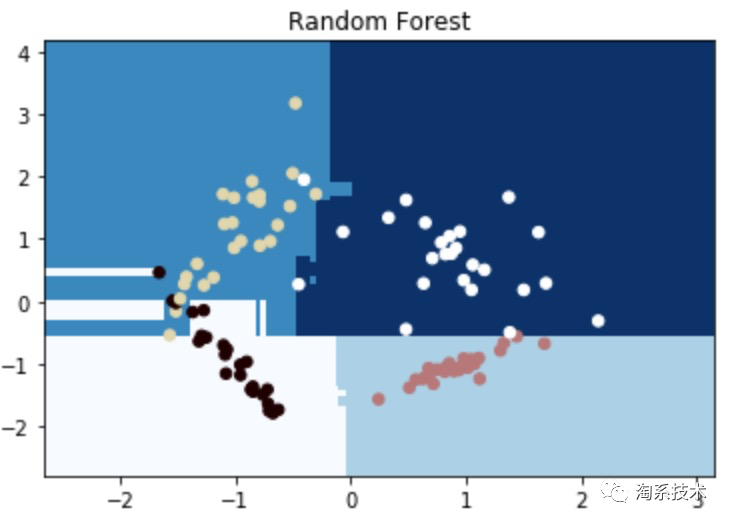import matplotlib.pyplot as plt
import numpy as np
from sklearn.model_selection import train_test_split
from sklearn.datasets import make_classification

# Decision Tree
from sklearn.tree import DecisionTreeClassifier
# 1. 准备数据
dt_X_train, dt_y_train = make_classification(n_features=2, n_redundant=0, n_informative=2,
random_state=1, n_clusters_per_class=1, n_classes=4)
# 2. 构造训练与测试集
l, r = dt_X_train[:, 0].min() - 1, dt_X_train[:, 0].max() + 1
b, t = dt_X_train[:, 1].min() - 1, dt_X_train[:, 1].max() + 1
n = 1000
grid_x, grid_y = np.meshgrid(np.linspace(l, r, n), np.linspace(b, t, n))
dt_X_test = np.column_stack((grid_x.ravel(), grid_y.ravel()))
# 3. 训练模型
dt_model = DecisionTreeClassifier(max_depth=4)
dt_model.fit(dt_X_train, dt_y_train)
# 4. 预测数据
dt_y_pred = dt_model.predict(dt_X_test)
# 5. 可视化
grid_z = dt_y_pred.reshape(grid_x.shape)
plt.figure('Decision Tree')
plt.title('Decision Tree')
plt.pcolormesh(grid_x, grid_y, grid_z, cmap='Blues')
plt.scatter(dt_X_train[:, 0], dt_X_train[:, 1], s=30, c=dt_y_train, cmap='pink')
plt.show()

▐  Support Vector Machines

它将数据映射为空间中的点，使得不同类别的点可以被尽可能宽的间隔分隔开，对于待预测类别的数据，先将其映射至同一空间，并根据它落在间隔的哪一侧来得到对应的类别。具体原来参考：看了这篇文章你还不懂SVM你就来打我 - SMON的文章 - 知乎，下面给出代码实现。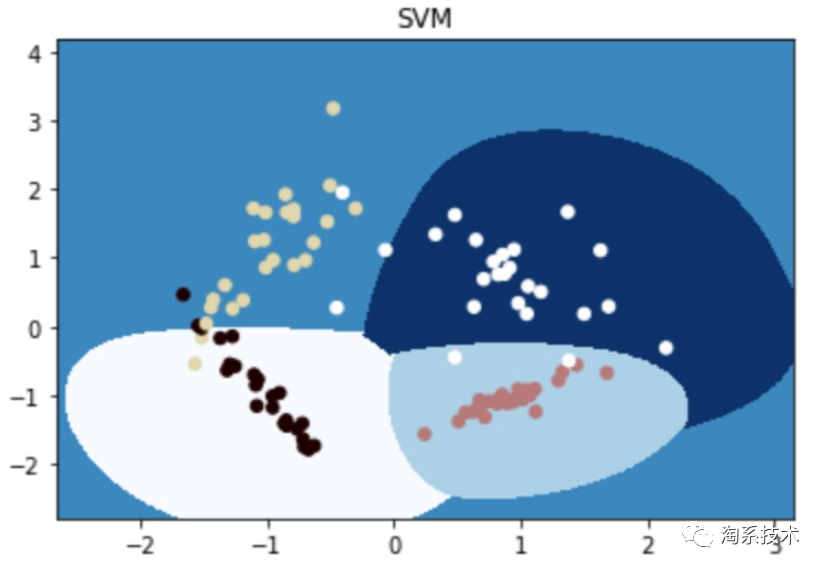import matplotlib.pyplot as plt
import numpy as np
from sklearn.model_selection import train_test_split
from sklearn.datasets import make_classification

# SVM
from sklearn import svm
# 1. 准备数据
svm_X_train, svm_y_train = make_classification(n_features=2, n_redundant=0, n_informative=2,
random_state=1, n_clusters_per_class=1, n_classes=4)
# 2. 构造训练与测试集
l, r = svm_X_train[:, 0].min() - 1, svm_X_train[:, 0].max() + 1
b, t = svm_X_train[:, 1].min() - 1,svm_X_train[:, 1].max() + 1
n = 1000
grid_x, grid_y = np.meshgrid(np.linspace(l, r, n), np.linspace(b, t, n))
svm_X_test = np.column_stack((grid_x.ravel(), grid_y.ravel()))
# 3. 训练模型
# svm_model = RandomForestClassifier(max_depth=4)
svm_model = svm.SVC(kernel='rbf', gamma=1, C=0.0001).fit(svm_X_train, svm_y_train)
svm_model.fit(svm_X_train, svm_y_train)
# 4. 预测数据
svm_y_pred = svm_model.predict(svm_X_test)
# 5. 可视化
grid_z = svm_y_pred.reshape(grid_x.shape)
plt.figure('SVM')
plt.title('SVM')
plt.pcolormesh(grid_x, grid_y, grid_z, cmap='Blues')
plt.scatter(svm_X_train[:, 0], svm_X_train[:, 1], s=30, c=svm_y_train, cmap='pink')
plt.show()

▐  K-Means

将数据划分到K个聚类簇中，使得每个数据点都属于离它最近的均值（即聚类中心，centroid）对应的集聚类簇。最终，具有较高相似度的数据对象划分至同一类簇，将具有较高相异度的数据对象划分至不同类簇。具体原理参考：用人话讲明白快速聚类kmeans - 化简可得的文章 - 知乎，下面给出代码的实现。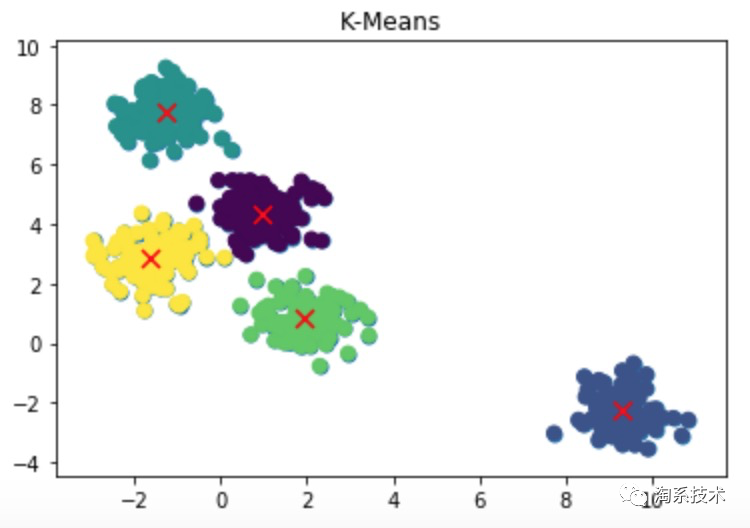import matplotlib.pyplot as pltimport numpy as npfrom sklearn.model_selection import train_test_splitfrom sklearn.datasets.samples_generator import make_blobs
# K-means 任务为聚类 n_classes=5from sklearn.cluster import KMeans
# 1. 准备数据kmeans_X_data, kmeans_y_data = make_blobs(n_samples=500, centers=5, cluster_std=0.60, random_state=0)# 2. 训练模型kmeans_model = KMeans(n_clusters=5)kmeans_model.fit(kmeans_X_data)# 3. 预测模型kmeans_y_pred = kmeans_model.predict(kmeans_X_data)# 4. 可视化plt.figure('K-Means')plt.title('K-Means')plt.scatter(kmeans_X_data[:,0], kmeans_X_data[:, 1], s=50)plt.scatter(kmeans_X_data[:, 0], kmeans_X_data[:, 1], c=kmeans_y_pred, s=50, cmap='viridis')centers = kmeans_model.cluster_centers_plt.scatter(centers[:,0], centers[:, 1], c='red', s=80, marker='x')plt.show()

▐  PCA

一种常用的降维技术，顾名思义，PCA帮助我们找出数据的主要成分，主成分基本上是线性不相关的向量，用选出的k个主成分来表示数据，来达到降维的目的。具体原理参考：如何通俗易懂地讲解什么是 PCA 主成分分析？- 马同学的回答 - 知乎，下面给出代码实现。

import matplotlib.pyplot as plt
import numpy as np
from sklearn.model_selection import train_test_split
from sklearn.datasets import make_classification

# PCA
from sklearn.decomposition import PCA

# 1. 准备数据
pca_X_data=pca_data.data
pca_y_data=pca_data.target
# 2. 训练模型, 维度为2
pca_model=PCA(n_components=2)
# 3. 降维
reduced_X=pca_model.fit_transform(pca_X_data)
# 4. 可视化
red_x,red_y=[],[]
blue_x,blue_y=[],[]
green_x,green_y=[],[]

for i in range(len(reduced_X)):
if pca_y_data[i] ==0:
red_x.append(reduced_X[i])
red_y.append(reduced_X[i])
elif pca_y_data[i]==1:
blue_x.append(reduced_X[i])
blue_y.append(reduced_X[i])
else:
green_x.append(reduced_X[i])
green_y.append(reduced_X[i])

plt.figure('PCA')
plt.title('PCA')
plt.scatter(red_x,red_y,c='r')
plt.scatter(blue_x,blue_y,c='b')
plt.scatter(green_x,green_y,c='g')
plt.show()

总结

至此，给出了常有的9种机器学习算法的实现，题主可以通过一些实际案例去进一步理解和熟悉算法。国外的Kaggle和阿里云天池都是获取项目经验的好途径。

推荐阅读：

https://zhuanlan.zhihu.com/p/72513104

https://zhuanlan.zhihu.com/p/139122386

https://www.cnblogs.com/pinard/p/6069267.html

https://www.cnblogs.com/pinard/p/6061661.html

https://zhuanlan.zhihu.com/p/26703300

https://zhuanlan.zhihu.com/p/51165358

https://zhuanlan.zhihu.com/p/49331510

https://zhuanlan.zhihu.com/p/75477709

淘系技术部-商业机器智能团队

商业机器智能部是一支数据和算法一体的团队，服务于淘宝、天猫、聚划算、闲鱼和躺平等业务线的二十余个业务场景，提供线上零售、内容社区、3D智能设计和端上智能等数据和算法服务。我们通过机器学习、强化学习、数据挖掘、机器视觉、NLP、运筹学、3D算法、搜索和推荐算法，为千万商家寻找商机，为平台运营提供智能化方案，为用户提高使用体验，为设计师提供自动搭配和布局，从而促进平台和生态的供给繁荣和用户增长，不断拓展商业边界。

这是一支快速成长中的学习型团队。在创造业务价值的同时，我们不断输出学术成果，在KDD、ICCV、Management Science等国际会议和杂志上发表数篇学术论文。团队学习氛围浓厚，每年组织上百场技术分享交流，互相学习和启发。真诚邀请海内外相关方向的优秀人才加入我们，在这里成长并贡献才智。

如果您有兴趣可将简历发至leihui.clh@alibaba-inc.com，期待您的加入！

✿  拓展阅读作者|陈雷慧（豆苗）

编辑|橙子君

出品|阿里巴巴新零售淘系技术展开全文算法 聚类 机器学习 人工智能 深度学习
• 前一篇文章普及了基于机器学习的恶意代码检测技术，主要参考郑师兄的视频总结，包括机器学习概述与算法举例、基于机器学习方法的恶意代码检测、机器学习算法在工业界的应用。这篇文章将分享两篇论文，介绍机器学习是...

您或许知道，作者后续分享网络安全的文章会越来越少。但如果您想学习人工智能和安全结合的应用，您就有福利了，作者将重新打造一个《当人工智能遇上安全》系列博客，详细介绍人工智能与安全相关的论文、实践，并分享各种案例，涉及恶意代码检测、恶意请求识别、入侵检测、对抗样本等等。只想更好地帮助初学者，更加成体系的分享新知识。该系列文章会更加聚焦，更加学术，更加深入，也是作者的慢慢成长史。换专业确实挺难的，系统安全也是块硬骨头，但我也试试，看看自己未来四年究竟能将它学到什么程度，漫漫长征路，偏向虎山行。享受过程，一起加油~

前一篇文章普及了基于机器学习的恶意代码检测技术，主要参考郑师兄的视频总结，包括机器学习概述与算法举例、基于机器学习方法的恶意代码检测、机器学习算法在工业界的应用。这篇文章将分享两篇论文，介绍机器学习是如何运用到恶意代码攻击中的，并谈谈自己的理解，后续深入研究尝试分享相关实验，目前还是小白一只。基础性文章，希望对您有所帮助，详见参考文献。

作者作为网络安全的小白，分享一些自学基础教程给大家，主要是在线笔记，希望您们喜欢。同时，更希望您能与我一起操作和进步，后续将深入学习AI安全和系统安全知识并分享相关实验。总之，希望该系列文章对博友有所帮助，写文不易，大神们不喜勿喷，谢谢！如果文章对您有帮助，将是我创作的最大动力，点赞、评论、私聊均可，一起加油喔！

前文推荐：

作者的github资源：

随着互联网的繁荣，现阶段的恶意代码也呈现出快速发展的趋势，主要表现为变种数量多、传播速度快、影响范围广。在这样的形势下，传统的恶意代码检测方法已经无法满足人们对恶意代码检测的要求。比如基于签名特征码的恶意代码检测，这种方法收集已知的恶意代码，以一种固定的方式生成特定的签名，维护这样的签名库，当有新的检测任务时，通过在签名库中检索匹配的方法进行检测。暂且不说更新、维护签名库的过程需要耗费大量的人力物力，恶意代码编写者仅仅通过混淆、压缩、加壳等简单的变种方式便可绕过这样的检测机制。

为了应对上面的问题，基于机器学习的恶意代码检测方法一直是学界研究的热点。由于机器学习算法可以挖掘输入特征之间更深层次的联系，更加充分地利用恶意代码的信息，因此基于机器学习的恶意代码检测往往表现出较高的准确率，并且一定程度上可以对未知的恶意代码实现自动化的分析。下面让我们开始进行系统的介绍吧~

一.什么是恶意代码？

恶意代码（Malicious Code） 是指运行在目标主机中，按照攻击者所规定逻辑执行的指令，其类别包括计算机病毒、蠕虫、木马、僵尸网络、勒索软件等。恶意代码攻击可以窃取核心数据和敏感信息，甚至对计算机系统和网络造成破坏，是当今网络安全的最大威胁之一。

恶意代码分析是一种解剖恶意代码的艺术，了解恶意代码是如何工作、如何识别，以及如何战胜或消除它。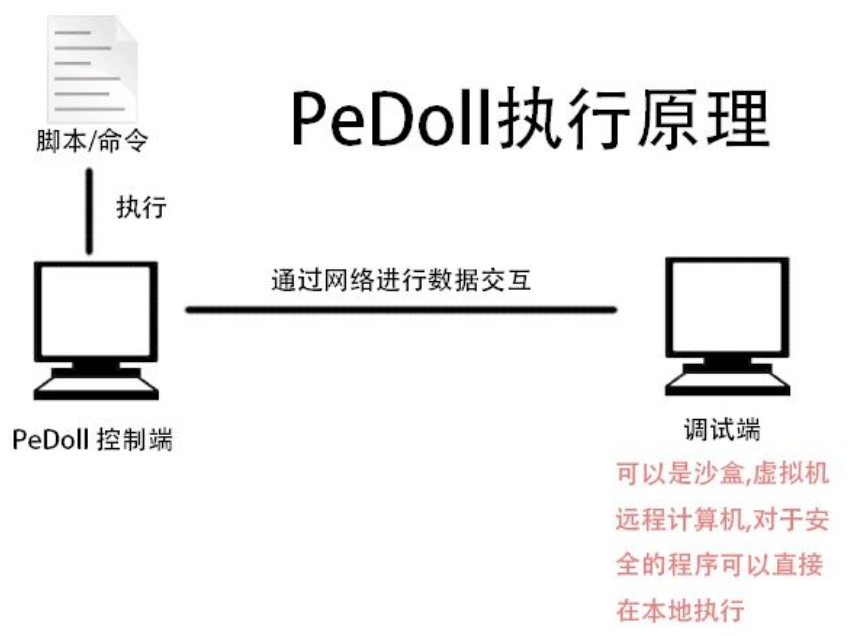基于行为的恶意代码检测技术 被许多安全厂商用来打造“主动防御”、“启发式查毒”产品。瑞星合理地将该技术应用于本机威胁感知、本机威胁化解及“云安全”中心威胁自动判定分析中，该技术是瑞星“云安全”策略实施的辅助支撑技术之一。

二.恶意代码检测方法

（一）传统的恶意代码检测

传统的恶意代码检测包括基于签名特征码 （ signature ）的检测和基于启发式规则（heuristic）的检测，在应对数量繁多的未知恶意代码时，正面临越来越大的挑战。

1.基于签名特征码的检测
签名特征码检测方法通过维护一个已知的恶意代码库，将待检测代码样本的特征码与恶意代码库中的特征码进行比对，如果特征码出现匹配，则样本为恶意代码。该方法需要耗费大量的人力、物力对恶意代码进行研究并要求用户及时更新恶意代码库，检测效率和效果越来越力不从心，并且很难有效抵御未知恶意代码。

2.基于启发式规则的检测
启发式规则检测方法通过专业的分析人员对现有的恶意代码进行规则提取，并依照提取出的规则对代码样本进行检测。但面对现阶段恶意代码爆炸式的增长趋势，仅依赖人工进行恶意代码分析，在实施上变得愈发困难。

传统的特征检测技术优缺点如下图所示：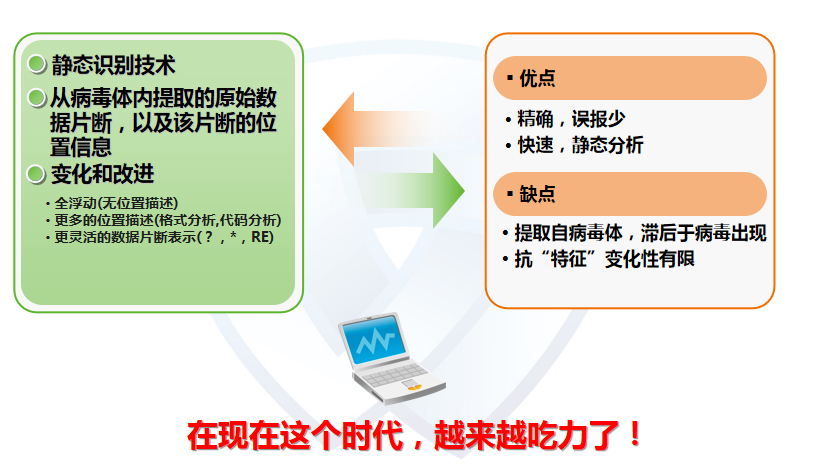那么，什么是特征码技术呢？行为分析又是指什么呢？

人类社会的“特征码”技术是——指纹。截取初犯的指纹放入档案，当再犯时，查对指纹，就可确定谁是犯人。法院可以根据法律和收集的信息来定罪。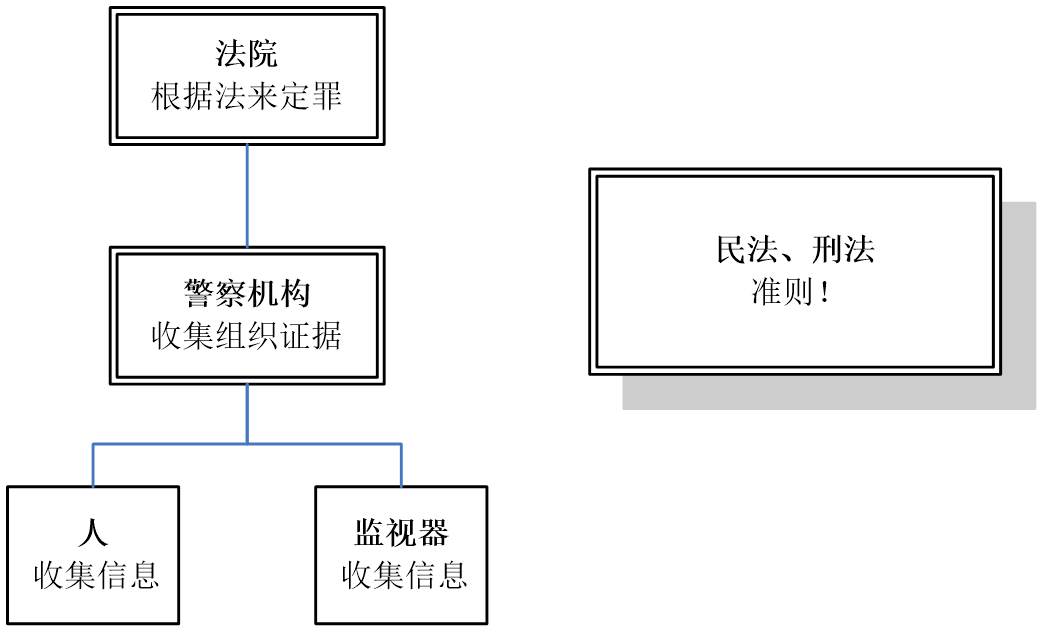我们又怎么给程序判定罪证呢？
把程序看成“人”，制定适用于这些“人”的“法律”，监视这个“人”的动作，整理、归纳收集到的信息，根据“法律”来判定“人”的好坏，行为分析就这样出现了！

行为分析定义为将一系列已经规定好的恶意行为做为规范，根据这些规范，去监视程序做了什么，再结合这个规范来判定程序是否是恶意代码。它并不什么新技术，而是病毒分析专家判定经验的应用。

瑞星公司的行为分析模型如下图所示，在恶意行为库中，监控层见识程序作了什么，组织层抽象信息，判断模块确定具体判定方式。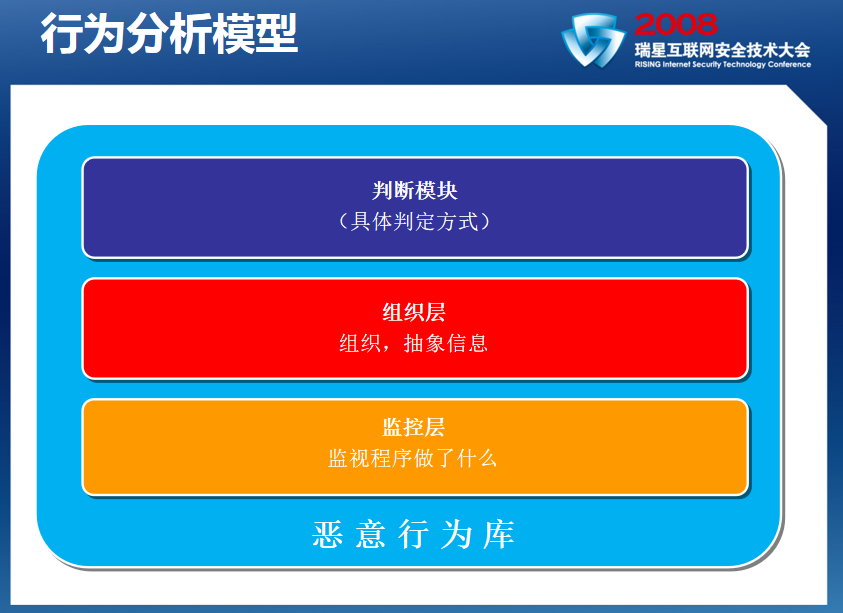通过推理机和恶意行为库判断恶意行为、恶意程序和正常程序。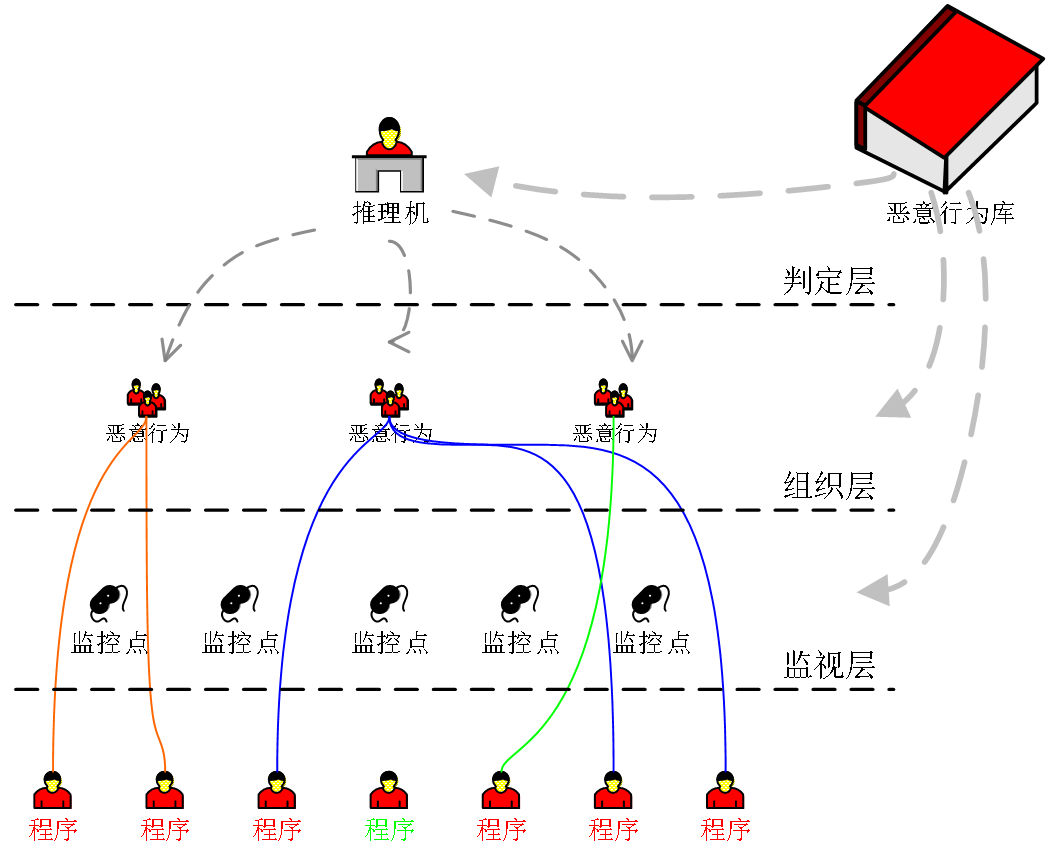恶意行为库是系统设计和实施的重点，直接影响整个系统的设计、实现以及效果。恶意动作、恶意行为要尽可能地区别正常程序与恶意代码，病毒分析经验的运用。除了病毒分析专家之外，没有再合适不过的人选了。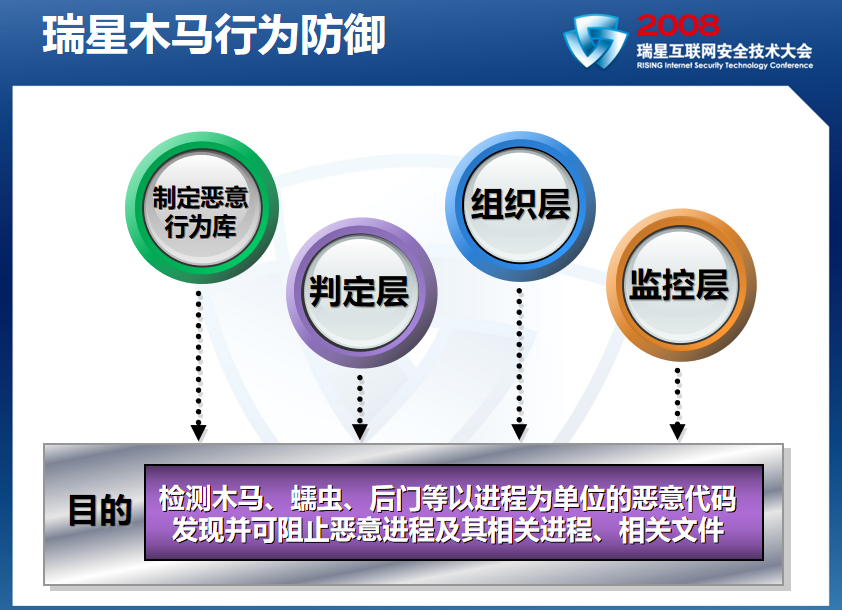木马行为防御的判定层实现：

• 针对进程集进行判定。
• 实时比对，为每个进程集合创建并维护恶意行为库的匹配上下文。
• 内置恶意动作发生即可，顺序无关。
• 扩展恶意动作按顺序判定。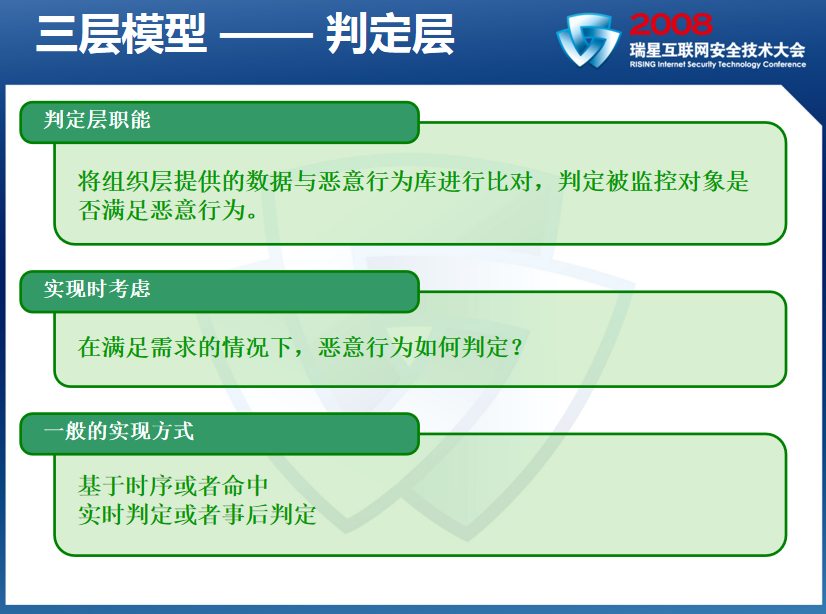木马行为防御的组织层实现：

• 相关进程集合（创建关系，释放关系）。
• 忽略可见进程的程序动作。
• 必要时将程序动作加工成恶意动作。
• 记录程序创建或修改的文件。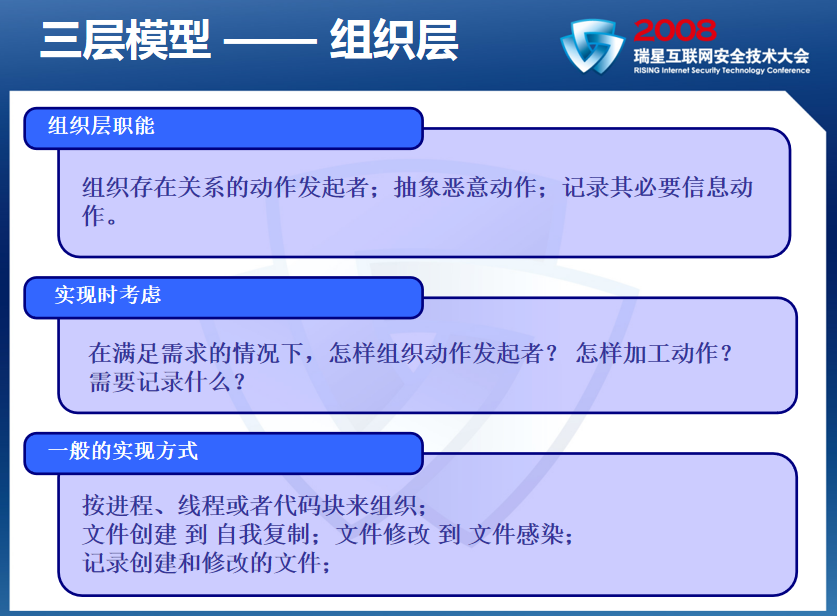木马行为防御的监控层实现：

• 文件监控。
• 进程监控。
• 注册表监控。
• 关键API调用监控。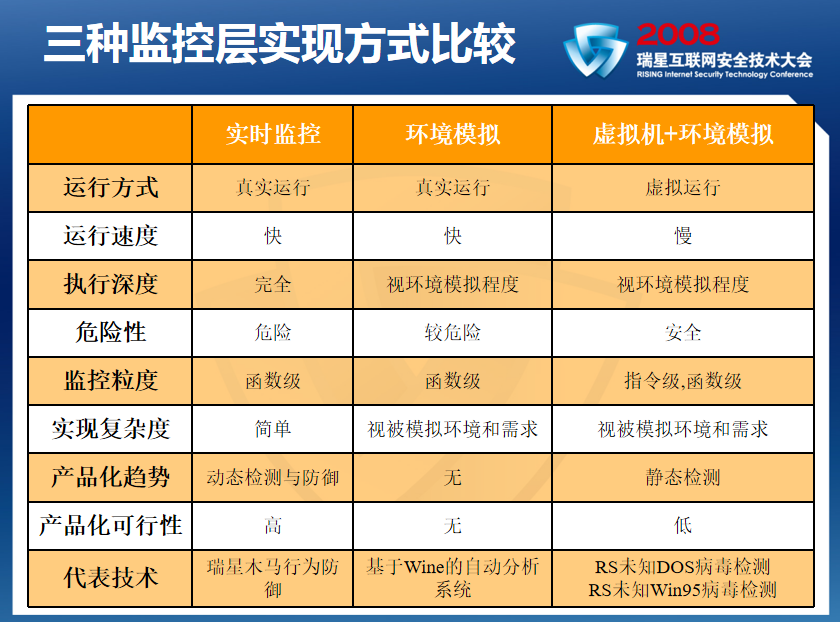指定恶意行为库：

• 恶意动作：（内置）自我复制，建立自启动关联，挂接全局自释放钩子等；（可扩展）程序动作+约束（自定义特征）。
• 恶意行为：多个不重复内置恶意动作，一组有先后顺序的扩展恶意动作。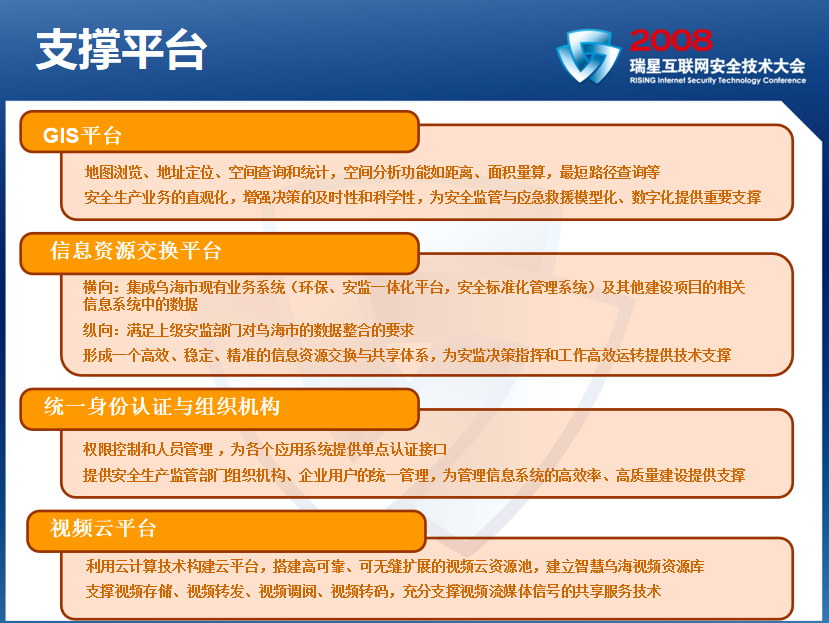未来做什么：

• 快速虚拟机实现
• 更合适规模的模拟环境实现
• 更细粒度的信息组织
• 更多的恶意动作（二）基于机器学习算法的恶意代码检测

基于机器学习算法的防护技术为实现高准确率、自动化的未知恶意代码检测提供了行之有效的技术途径，已逐渐成为业内研究的热点。根据检测过程中样本数据采集角度的不同，可以将检测分为：静态分析与动态分析

静态分析不运行待检测程序，而是通过程序（如反汇编后的代码）进行分析得到数据特征，而动态分析在虚拟机或仿真器中执行程序，并获取程序执行过程中所产生的数据（如行为特征），进行检测和判断。

根据 Cohen 对恶意代码的研究结果，可知恶意代码检测的本质是一个分类问题，即把待检测样本区分成恶意或合法的程序。
其核心步骤为：

1. 采集数量充分的恶意代码样本；（难点）
2. 对样本进行有效的数据处理，提取特征；（难点）
3. 进一步选取用于分类的主要数据特征；
4. 结合机器学习算法的训练，建立分类模型；
5. 通过训练后的分类模型对未知样本进行检测。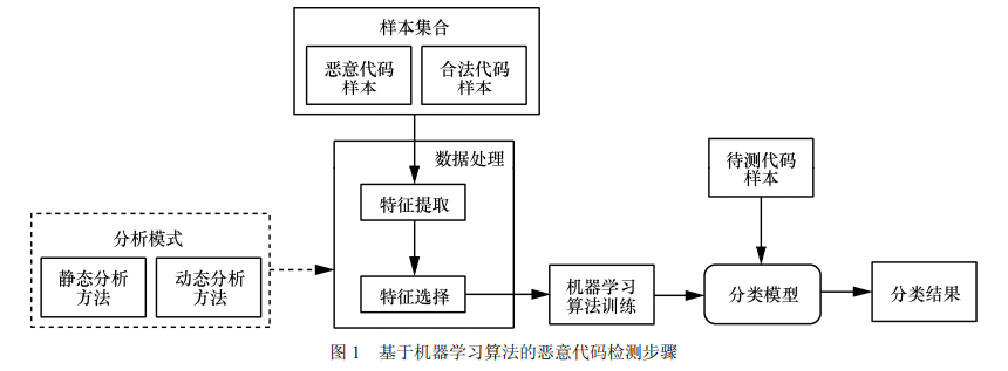三.恶意代码样本采集

恶意代码样本的有效采集是进行代码分析工作的基础。当结合机器学习算法进行检测时，只有通过充分的样本数据训练，分类模型才能更准确地实现检测功能。一般来讲，恶意代码样本的获取途径有如下几种。

1.用户端采样
这是大多数杀毒软件厂商的主要获取方法，使用杀毒软件的终端用户将恶意代码样本上传至厂商。该方法具有较好的实时性，但安全厂商的样本数据往往选择不对外开放，很难直接获取。

2.公开的网络数据库
如 VirusBulletin、Open Malware、VX Heavens等，相比恶意代码的更新速度，现阶段公开在线样本系统较有限，且站点存在隐蔽性不足、易遭到攻击的问题。因此，建立威胁情报的共享机制，日益突显出其重要性。

3. 其他技术途径
通过蜜罐（如 Nepenthes蜜罐）等捕获工具进行搜集，即设计一个专门的具有脆弱性的系统，诱导攻击者进行攻击进而得到恶意代码样本。一些木马和网络后门等也可以通过垃圾邮件陷阱或安全论坛（如卡饭论坛）的方式得到。
不过，上述技术途径的捕获样本规模较有限。

蜜罐技术本质上是一种对攻击方进行欺骗的技术，通过布置一些作为诱饵的主机、网络服务或者信息，诱使攻击方对它们实施攻击，从而可以对攻击行为进行捕获和分析，了解攻击方所使用的工具与方法，推测攻击意图和动机，能够让防御方清晰地了解他们所面对的安全威胁，并通过技术和管理手段来增强实际系统的安全防护能力。

四.基于机器学习的静态分析方法

提取恶意代码的静态特征，通过对程序代码进行逆向分析。常用的工具包括 IDA Pro、Hopper、OllyDbg 等。

1.样本特征提取
①基于序列的特征类型
该方法在样本特征的提取上应用最为广泛，其代表技术为 N 元语法模型（N-gram）。N-gram 假定 N 个出现的词只与之前出现的 N−1 个词相关，其中，N 代表一个特征序列的长度。如果考虑一个长为 L 的词组集合，则 N元语法模型会通过滑动窗口的形式，将词组划分为 L−N+1 个特征序列。例如，当 3-gram 被应用在词集{PUSH, SUB, SAL, AND, DIV, LDS, POP}上（此时 L=7）时，如图 2 所示，会得到 5 个特征序列，每个序列包含 3 个词元。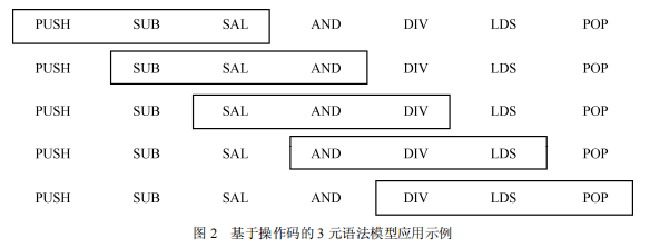Abou-Assaleh 等首先提出了基于字节（Byte）序列的特征提取框架，并使用 K 近邻分类方法实现了恶意代码的有效检测。另一类词元选择方式是基于操作码（Opcode）的，Opcode是描述程序执行操作的机器语言指令，相对于字节序列来讲，具有更强的实际意义和可靠性，结合 Opcode 的特征提取可以更好地表征恶意代码。基于Opcode序列能完成了对恶意代码进化的追踪，Siddiqui等结合操作码序列的方式，通过神经网络、决策树等分类算法，实现了 98.4%的检测准确率。

②基于字符串的特征类型
另一种特征类型的提取方式是基于程序代码中的可输出字符串，因为可输出字符串在某种程度上反映了待测程序的意图。例如，从代码中的“https://…”字符串可以推测程序的网络连接意图；而包含目录路径的字符串则说明程序可能尝试读取用户文档或注册表信息等。文献选取了可执行文件中 100 个可输出字符串，以此为特征训练基于支持向量机的分类器，实现了99.38%的准确率。与基于序列的特征类型相比，代码中的字符串数量有限，因此提取的特征集具有较少的维度，在计算成本上可以实现有效的控制。

③基于 API 调用的特征类型
程序对应用程序编程接口（API，application programming interface）调用也可以作为特征类型。文献对 API 调用进行了讨论，指出程序PE（portable executable）文件头中的 API 信息不具有准确性，因为恶意代码会在 PE 文件头中夹杂错误的 API 信息。Ding等对反汇编后的代码进行 API 调用分析，利用恶意代码和合法代码应用程序编程接口分布的差异性提取了基于 API 调用的程序特征。文献将代码中的 API 调用序列转化为对应的马尔可夫（Markov）链，有向图中边的权重表示调用 API 的状态转移概率，通过基于 Markov 链的特征提取，实现了对未知恶意软件的有效分类。

2.样本特征选取
由于提取的数据特征常包含冗余信息，容易引起过度拟合问题，本节对数据特征选取的主要方法进行介绍，其种类主要包括信息增益（IG，information gain）、增益率（GR，gainratio）、文档频率（DF，document frequency）、主成分分析（PCA，principal component analysis）等。主成分分析也是一类常见的特征选取方法，在静态、动态分析中常被用于实现对样本数据的降维。PCA 通过线性变换，将原始数据投射到新的坐标系下，并通过新空间中最大线性无关组对数据样本进行表达，该线性无关组特征值的空间坐标即 PCA 方法所选取的特征。与 IG、DF 等方法不同，PCA 使用变换后的特征，而非原始特征的子集。

五.基于机器学习的动态分析方法

恶意代码的静态分析技术，在应对代码混淆或加壳等情形时，具有一定的局限性。为了保证代码评估的准确性，动态分析技术利用虚拟机或仿真器执行待测程序，监控并收集程序运行时显现的行为特征，并根据特征数据实现恶意代码的分类。

静态分析与动态分析区别：
调试逆向分为动态分析技术和静态分析技术。动态分析技术指的是使用调试工具加载程序并运行，随着程序运行，调试者可以随时中断目标的指令流程，以便观察相关计算的结果和当前的设备情况。静态分析技术是相对于动态分析而言的。由于在实际分析中，很多场合不方便运行目标（例如病毒程序，设备不兼容，软件的单独某一模块）。那么这个时候就需要应用静态分析技术。OD（OllyDbg）和IDA Pro这两款工具分别是调试逆向的倚天剑和屠龙刀。虽然两者都兼容动态和静态的调试方式，但就动态调试而言，OD更为灵活和强大，而静态调试工具的王者理所应当是功能极为强大的IDA Pro。

1.行为特征提取
沙箱技术是收集行为特征的重要技术途径，许多安全公司提供了 Web 版的沙箱接口，用以对上传的程序样本进行动态分析，生成行为分析报告。目前常见的沙箱工具有 Anubis、Joe Sandbox、Cuckoo Sandbox、CWSandbox 等。

动态分析的重点是对监控行为的类型进行合理选择。一般来讲，基于行为分析的方案主要考察程序运行过程中所涉及的以下方面：

• 系统文件的改变，如创建或修改文件；
• 注册表键值的操作行为；
• 进程访问的情况；
• 系统服务行为，如开启、创建或删除服务；
• 网络访问情况；
• 应用程序编程接口（API）的调用。

此外，一些解决方案还对程序调用函数的数据信息进行分析，这时污点标签设置方法常被结合使用。

文献[22,23]结合行为报告的分析结果，对恶意代码的行为特征进行识别，借助机器学习算法对可执行文件进行分类。杨轶等通过分析污点传播的过程，识别不同的恶意代码行为间控制指令和数据的依赖关系，从而比较恶意代码的相似性。Imran 等通过隐马尔可夫模型对待测样本的动态行为特征进行描述，并借助机器学习算法实现分类。Anderson 等则通过动态方式搜集程序指令序列，进而生成基于马尔可夫链的有向图。

2.行为特征选取
许多沙箱工具，如 Anubis 和 CWSandbox 的输出格式为文本或可扩展标记语言（XML，
extensible markup language），这两类格式更适用于小规模样本的人工分析。具体来说，文本格式报告对行为特征的刻画过于简单，粒度较粗，一些重要的行为不再可见；而 XML 格式下的分析报告表述又过于繁冗，不便于开展自动化分析。为了高效处理行为分析数据，Trinius 等提出基于恶意软件指令集（MIST，malware instruction set）的行为数据描述方法，常被用来对其他格式（如 XML 格式）的行为报告进行转换，从而达到在行为数据中选取主要特征的目的。MIST 将程序行为的监控结果描述为一系列指令，其中每个线程和进程的执行流被分组在一个连续的报告中。每条指令都对应监控到的一个系统调用（system call）及其调用到的参数（argument），指令以短数值的方式予以标识。此外，系统调用的具体参数被分隔在不同等级的块中，反映不同程度的行为粒度。

MIST 报告可以进一步通过向量空间模型（VSM，vector space model）进行向量化，生成可用于机器学习算法分类的数据。在特征项和特征项权重的计算上，可运用词袋模型（BOW，bag of words）。

词袋模型的示例如下，假设有下述 2 个文件。

1. Samuel detected a malware. I detected the malware too.
2. The malware was detected by us.

基于上述 2 个文件，可以构建一个词汇表。
词汇表={1．“Samuel”，2．“detected”，3．“a”， 4．“malware”，5．“I”，6．“the”，7．“too”，8．“was”， 9．“by”，10．“us”}。

这个词汇表一共包含 10个不同的单词，利用索引号，上面 2 个文件可分别用 10 维向量表示（向量中元素为词表单词在文件中出现的频率）。


[0，l，01010111]

利用词袋模型，经过 MIST 处理后的指令语句将作为 VSM 模型中的特征项，指令的出现频率即为特征项的权重，以建立恶意代码的向量空间数据，这样就可以利用机器学习算法（如支持向量机）进行恶意代码的分类。

六.恶意代码分类算法

恶意代码进行静态、动态分析后得到的特征数据，可以作为机器学习算法训练的输入，产生
相应的恶意代码分类器。常见的算法如 K近邻（KNN，k nearest neighbor）、支持向量机
（SVM，support vector machine）、朴素贝叶斯（Naïve Bayes）、决策树（DT，decision tree）、随机森林（RF，randomforest），以及深度学习算法，如卷积神经网络（CNN，convolutional neural network）等。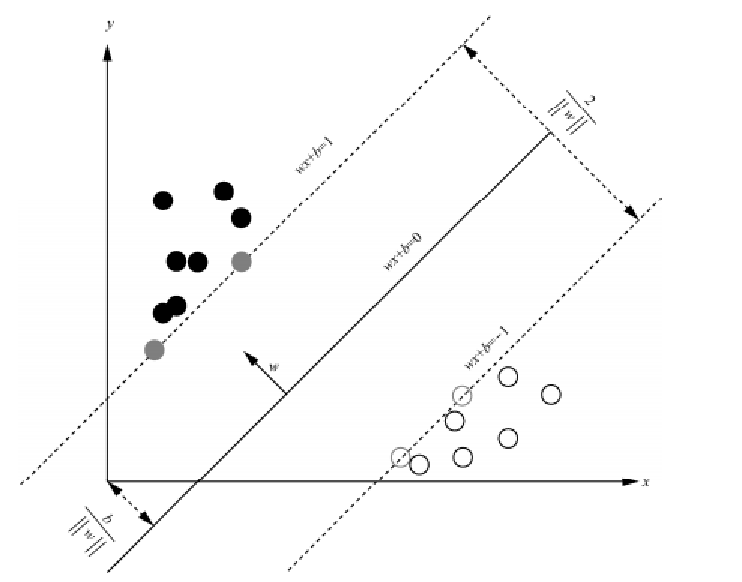下图展示了一种投毒攻击的示意图，以及机器学习训练过程中安全威胁及防御措施。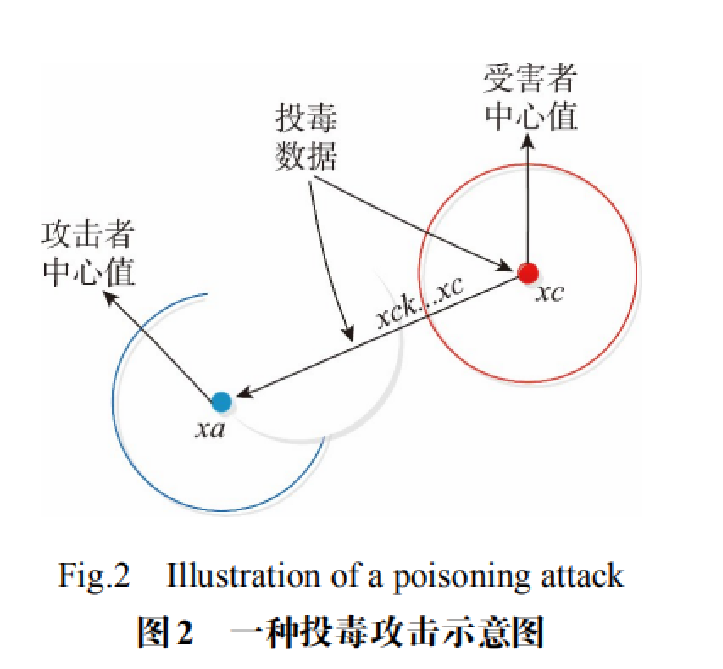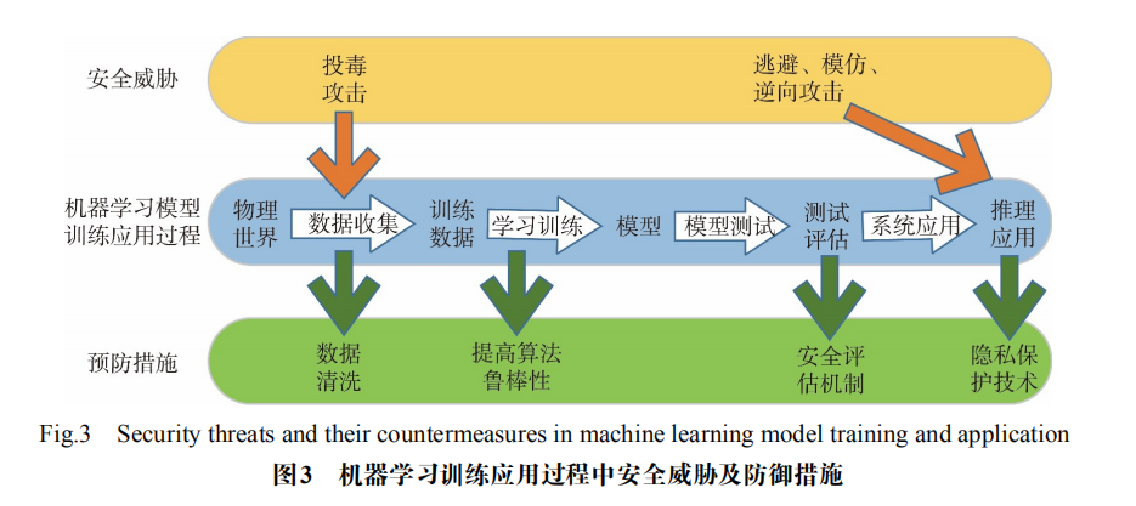在考虑敌手视角时，如果攻击者也通过机器学习技术优化恶意代码的设计，对攻击目标画像并实现精准攻击，该如何应对？同时，又该如何保证机器学习引擎不被攻击者“投毒”，防止出现干扰项致使训练出错产生误判，这些都是需要进一步研究和思考的问题。

七.恶意代码检测实战知识

下面简单举一个示例——冰河木马分析与检测。后续希望自己能深入学习，学会这些实例分析，加油！

冰河开发的最初原因是为了开发一个功能强大的远程控制软件。但一经推出就成了黑客们的入侵工具。2006年以前冰河一直是国内不动摇的领军木马。功能有自动跟踪目标机屏幕变化、记录各种口令信息、获取系统信息、限制系统功能、远程文件操作、远程文件操作等。下面从以下几个不同方面分析冰河木马。

1.进程检测
从Procexp软件可以明显的看到，有一个KERNEL32.EXE进程（能否进一步确定该进程调用的模块，进一步找准木马程序）。这个明显是假装系统进程的木马进程，CPU使用率达到了99%！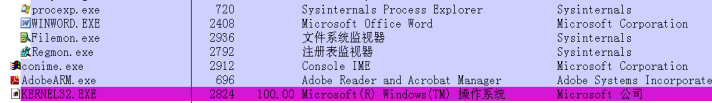2.文件监测
用Filemon监测到，样本先在c:\Windows\system32 目录创建了一个KERNEL32.EXE文件，并往其中写入了大量与自身运行有关的数据。如下图所示：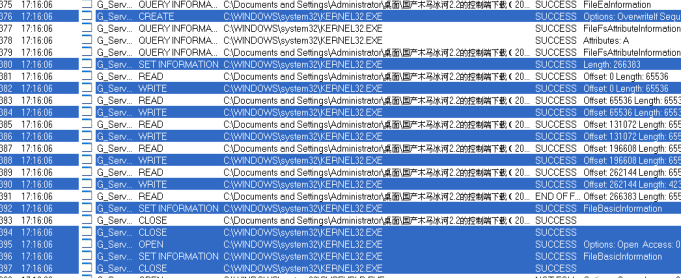然后又在C:\Windows\system32目录下创建一个名SYSEXPLR.EXE的文件，随后又把查看了电脑文件目录信并把它们写入这两个文件。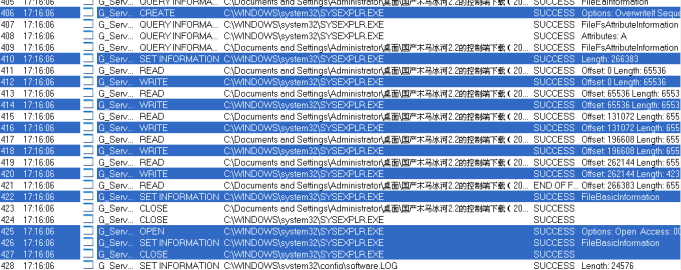3.注册表监测
从Regmon我们可以看出，木马把KERNEL32.EXE注册成了服务。并把KERNEL32.EXE注册为开机启动。
HKLM\Software\Microsoft\Windows\CurrentVersion\RunServices(Default) SUCCESS “C:\WINDOWS\system32\KERNEL32.EXE”
如下图所示：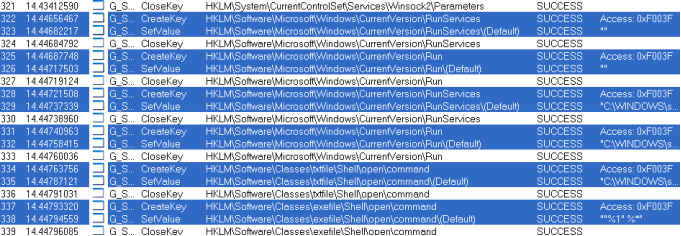另外，木马还修改了 .TXT 文件的关联， sysexplr.exe和TXT文件关联。即使删除了Kernel32.exe，但只要你打开 TXT文件，sysexplr.exe就会被激活，它将再次生成Kernel32.exe，于是冰河又回来了。

4.系统通信端口监测
通过TCPView监测 KERNEL32.EXE开启了TCP7626端口。如下图所示：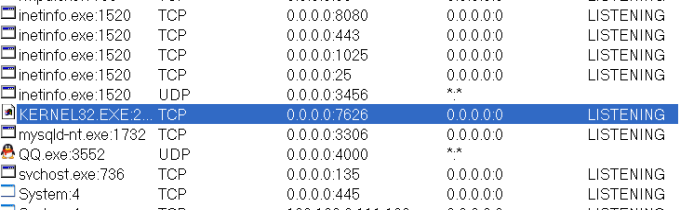接着需要分析木马样本外部特征，包括文件特征、注册表特征、进程特征、端口特征等。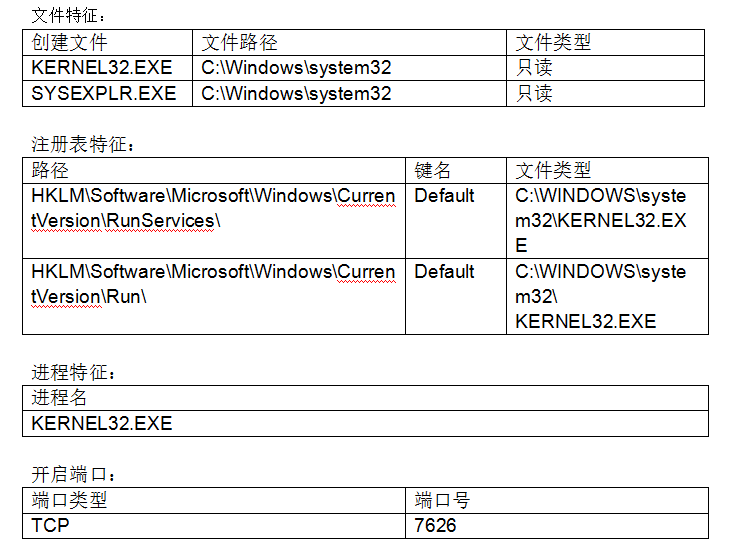该木马的清除方法如下：

• 删除C:Windows\system下的Kernel32.exe和Sysexplr.exe文件。
• 删除注册表HKEY_LOCAL_MACHINE/software/microsoft/windows/
CurrentVersionRun下键值为C:/windows/system/Kernel32.exe。
• 删除注册表HKEY_LOCAL_MACHINE/software/microsoft/windows/ CurrentVersion/Runservices下键值为C:/windows/system/ Kernel32.exe。
• 最后改注册表HKEY_CLASSES_ROOT/txtfile/shell/ open/command 下的默认值，由中木马后的C: /windows/system/Sysexplr.exe %1改为 正常情况下的C:/windows /notepad.exe %1，即可恢复TXT文件关联功能。

八.总结

在网络攻击日益复杂、恶意代码层出不穷的今天，机器学习算法在恶意代码检测中的应用逐渐受到学术界和众多安全厂商的重视。本文对基于机器学习算法恶意代码检测的技术方法和主流方案进行了梳理和讨论，这一工作将为新型主机恶意代码检测技术的设计和实现提供重要参考。但该领域仍属于发展阶段，还存在着许多未来工作和挑战，对其归纳如下。

• 静态分析检测速度快、系统资源占用少，但随着代码混淆、加壳等反检测技术的发展，静态分析的准确性受到一定程度的限制。动态分析技术需要运行被测代码，在效率上存在局限性。一个主流的发展方向是将静态、动态分析技术进行有效结合，全方位地对待测代码进行评估。
• 机器学习算法可以提供高准确率的恶意代码分类，但分类器一般作为黑盒机制被加以使用，安全人员缺乏对结果的理解。结果往往在不质疑分类器性质的情况下直接被使用，因此分类结果受经验阈值和数据特征的影响，出现一定倾向性。研究传统量化分析（如准确率、误报率）之外的统计学方法，如可信度（credibility），科学地评价和比较底层的机器学习算法，是未来一项重要的研究工作。最后希望基础性文章对您有所帮助，作者也是这个领域的菜鸟一枚，希望与您共同进步。

(By:Eastmount 2021-09-27 晚上10点夜于武汉 http://blog.csdn.net/eastmount/ )

参考文献如下，本文参考了这些内容，再次感谢这些大佬。

•  张东, 张尧, 刘刚, 宋桂香. 基于机器学习算法的主机恶意代码检测技术研究[J]. 网络与信息安全学报, 2017(7): 25-32.
•  杨轶, 苏璞睿, 应凌云, 等. 基于行为依赖特征的恶意代码相似性比较方法[J]. 软件学报, 2011, 22(10): 2438-2453.
•  杨晔. 基于行为的恶意代码检测方法研究[D]. 西安: 西安电子科技大学, 2015.
•  李盼，赵文涛，刘强+，崔建京，殷建平. 机器学习安全性问题及其防御技术研究综述，计算机科学与 探索，2018(12).
•  张蕾, 崔勇, 刘静, 江勇, 吴建平. 机器学习在网络空间安全研究中的应用[J]. 计算机学报, 2018(9): 1943-1975.
•  IMRAN M, AFZAL M T, QADIR M A. Malware classification using dynamic features and hidden markov model[J]. Journal of In telligent & Fuzzy Systems, 2016, 31(2):837-847.
•  恶意程序行为分析工具 PeDoll - DBinary
•  《恶意代码分析实战》诸葛建伟 姜辉 张光凯
•  https://www.cnblogs.com/yunji5566/p/4249927.html
•  2008年瑞星安全技术大会
展开全文人工智能 机器学习 网络安全 AI安全
• 机器学习算法+代码

千次阅读 2021-11-19 20:55:48
机器学习 一、概述 1、机器学习研究方向 传统预测 图像识别 自然语言处理 2、数据集构成 数据集 = 特征值+目标值 监督学习： 目标值为类别 属于分类问题 目标值为连续数据 属于回归问题 无监督学习 无目标值 3...

一、概述

• 传统预测
• 图像识别
• 自然语言处理

2、数据集构成

数据集 = 特征值+目标值

监督学习：

• 目标值为类别 属于分类问题
• 目标值为连续数据 属于回归问题

无监督学习

• 无目标值

3、机器学习流程

1. 获取数据
2. 数据处理
3. 特征工程
4. 机器学习模型-训练模型
5. 模型评估
6. 应用

4、书籍、框架

机器学习深度学习
数集西瓜书–周志华花书
框架sklearntheano、caffe2、chainer
数据集sklearn、kaggle、UCI

Kaggle网址：https://www.kaggle.com/datasets

UCI数据集网址： http://archive.ics.uci.edu/ml/

scikit-learn网址：[http://scikit-learn.org/stable/datasets/index.html#datasets]

二、特征工程

1、数据集

pip3 install Scikit-learn==0.19.1

from sklearn.datasets import fetch_**#大数据集

#鸢尾花数据集

#波士顿房价数据集

2、大规模数据集

datasets.fetch_*(data_home=None,subset=“train”)

• data_home，表示数据集下载的目录,默认是 ~/scikit_learn_data/
• subset:train表示只要测试集；all表示全部数据都要；test表示只要测试集

3、数据集的返回值

返回值类型：datasets.base.Bunch（继承自字典）

返回值属性：

• data:特征值
• target:标签
• descr:数据描述
• feature_names:特征名
• target_names:标签名

调用返回值方式：

bunch.key = value

dict[‘key’] = value

def datasets_demo():
"""
sklearn数据集的使用
:return:
"""
# 获取数据集
print("鸢尾花数据集： \n" ,iris)
print("查看数据集描述： \n",iris['DESCR'])
print("查看数据集特征值的名字： \n",iris.feature_names)
print("查看数据集标签的名字： \n",iris.target_names)
print("查看数据集特征值： \n",iris.data.shape , iris.data)# shape：(150, 4)

return None

if __name__ == "__main__":
# 代码1：sklearn数据集使用
datasets_demo()

4、数据集划分

from sklearn.model_selection import train_test_split

机器学习一般的数据集会划分为两个部分：

• 训练数据：用于训练，构建模型
• 测试数据：在模型检验时使用，用于评估模型是否有效
sklearn.model_selection.train_test_split(arrays, *options)

• x 数据集的特征值

• y 数据集的标签值

• test_size 测试集的大小，一般为float

• random_state 随机数种子,不同的种子会造成不同的随机采样结果。相同的种子采样结果相同。

• return 测试集特征值、训练集特征值，训练标签（目标值），测试标签(目标值)

x_train , x_test , y_train , y_test

from sklearn.model_selection import train_test_split

def datasets_demo():
"""
sklearn数据集的使用
:return:
"""
# 获取数据集
#     print("鸢尾花数据集： \n" ,iris)
#     print("查看数据集描述： \n",iris['DESCR'])
#     print("查看数据.集特征值的名字： \n",iris.feature_names)
#     print("查看数据集数据： \n",iris.data.shape , iris.data)# shape：(150, 4)

# 数据集的划分
x_train,x_test,y_train,y_test = train_test_split(iris.data,iris.target,test_size=0.2,random_state=22 )
print("训练集的特征值： \n",x_train,x_train.shape)

return None

if __name__ == "__main__":
# 代码1：sklearn数据集使用
datasets_demo()

2、特征工程

什么是特征工程？

专业背景知识和技巧处理数据，使得特征能在机器学习算法上发挥更好的作用的过程。（使用pandas、numpy对特征进行些处理）

特征工程内容分为三类：特征抽取、特征预处理、特征降维

1、特征抽取/特征提取

1）字典特征提取(特征离散化)

from sklearn.feature_extraction import DictVectorizer

属性

• DictVectorizer.fit_transform(X) X:字典或者包含字典的迭代器返回值：返回sparse矩阵(稀疏矩阵)
• DictVectorizer.inverse_transform(X) X:array数组或者sparse矩阵 返回值:转换之前数据格式
• DictVectorizer.get_feature_names() 返回类别名称

使用场景

• 数据类型是字典
• 数据集中存在类别特征比较多（性别、等级），先将数据集的特征转化为字典类型，然后使用DictVerctorizer进行转换

代码

from sklearn.feature_extraction import DictVectorizer

def dict_demo():
"""
字典特征抽取
:return:
"""
data = [{'city': '北京', 'temperature': 100}
, {'city': '上海', 'temperature': 60}
, {'city': '深圳', 'temperature': 30}]

#     1、实例化转换器
transfer = DictVectorizer(sparse=False)
# 2、调用fit_transform
data_new = transfer.fit_transform(data,y=None)

print("打印返回结果： \n",data_new)

if __name__ == "__main__":
dict_demo()

sparse = False

[外链图片转存失败,源站可能有防盗链机制,建议将图片保存下来直接上传(img-3YarFVus-1637326542934)(https://i.loli.net/2021/11/06/fuSsliOqzFD9UVb.png)]

sparse=Ture,只表示不是0的东西，可节约内存

[外链图片转存失败,源站可能有防盗链机制,建议将图片保存下来直接上传(img-JHZcLEEz-1637326542936)(https://i.loli.net/2021/11/06/VOrXlQ3s8RjC9tG.png)]

注意：数据提取类似之前pandas中的one-hot编码

2）、文本特征提取

sklearn.feature_extraction.text import CountVectorizer

1、实例化

trans = CountVectorizer(stop_words)

stop_words表示停用词,即使样本中出现但是依旧不计数的词，通常有一个停用词表。

2、方法

• CountVectorizer.fit_transform(X)

X:文本或者包含文本字符串的可迭代对象 返回值：返回sparse矩阵

统计每个样本中特征值出现的次数

• CountVectorizer.inverse_transform(X)

X:array数组或者sparse矩阵 返回值:转换之前数据格

• CountVectorizer.get_feature_names() 返回值:单词列表

3、流程

• 实例化类CountVectorize
• 调用fit_transform方法输入数据并转换，注意返回格式，利用toarray()进行sparse矩阵转换array数组
sklearn.feature_extraction.text import CountVectorizer

def text_count_demo():
"""
对文本进行特征抽取，countvetorizer
:return:
"""
data = ["life is short,i like like python"
, "life is too long,i dislike python"]

# 1 实例化转换器类
transfer = CountVectorizer(stop_words=['is','like'])#stop_words:停用词

# 2 调用转换器中的方法
data_new = transfer.fit_transform(data)

print("data_new:\n",data_new.toarray())
print("array数组:\n",transfer.inverse_transform(data_new))
print("特征名字:\n",transfer.get_feature_names())

if __name__ == "__main__":

text_count_demo()

[外链图片转存失败,源站可能有防盗链机制,建议将图片保存下来直接上传(img-PGkO5UzW-1637326542937)(https://i.loli.net/2021/11/07/tysLV4nuAQFwo5j.png)]

注意：此方法不支持中文，因为中文中没有空格，除非先分词

4、jieba中文分词

pip3 install jieba

from sklearn.feature_extraction.text import CountVectorizer
import jieba

def china_text_count_demo():

"""
对中文文本进行特征抽取，countvetorizer
:return:
"""
data = ["我爱北京天安门"
, "天安门上太阳升"]

# 1 实例化转换器类
transfer = CountVectorizer()

# 2 调用转换器中的方法
data_new = transfer.fit_transform(data)

print("data_new:\n",data_new.toarray())
print("array数组:\n",transfer.inverse_transform(data_new))
print("特征名字:\n",transfer.get_feature_names())

def cut_word(text):
"""
进行中文分词“我爱北京天安门 --> 我 爱 北京 天安门”
:return:
"""

a = " ".join(list((jieba.cut(text))))
print(a)
return text

def china_text_count_demo_2():
"""
中文文本特征值化，自动分词
:return:
"""
# 1 将中文文本进行分词
data = ["一种还是一种今天很残酷，明天更残酷，后天很美好，"
"但绝对大部分是死在明天晚上，所以每个人不要放弃今天。",
"我们看到的从很远星系来的光是在几百万年之前发出的，"
"这样当我们看到宇宙时，我们是在看它的过去。",
"如果只用一种方式了解某样事物，你就不会真正了解它。"
"了解事物真正含义的秘密取决于如何将其与我们所了解的事物相联系。"]
data_new = []
for sent in data:
data_new.append(cut_word(sent))
# print(data_new)

# 2 实例化countvetorizer
transf = CountVectorizer()

# 3 调用fit_transform()
data_final = transf.fit_transform(data)
print("data_final: \n",data_final)
print("特征提取结果:\n",data_final.toarray())
print("特征名字:\n",transf.get_feature_names())
return None

if __name__ == "__main__":

# 代码5 中文文本特征值提取，自动分词
china_text_count_demo_2()

# 代码6  中文分词
# cut_word("我爱北京天安门")

5、Tf-idf 提取特殊高频词（关键词）

from sklearn.feature_extraction.text import TfidfVectorizer

• 如果某个词或者短语在一篇文章中出现频率很高但是在其他文章中出现很少，那么这个词可以视为关键字
• Tf-idf用以评估一字词对于一个文件集或一个语料库中的其中一份文件的重要程度

定义

• 词频（term frequency，tf）指的是某一个给定的词语在该文件中出现的频率
• 逆向文档频率（inverse document frequency，idf）是一个词语普遍重要性的度量。某一特定词语的idf，可以由总文件数目除以包含该词语之文件的数目，再将得到的商取以10为底的对数得到
from sklearn.feature_extraction.text import TfidfVectorizer
import jieba

def tfidf_demo():
"""
tfidf进行文章特征提取
:return:
"""
# 1 将中文文本进行分词
data = ["一种还是一种今天很残酷，明天更残酷，后天很美好，"
"但绝对大部分是死在明天晚上，所以每个人不要放弃今天。",
"我们看到的从很远星系来的光是在几百万年之前发出的，"
"这样当我们看到宇宙时，我们是在看它的过去。",
"如果只用一种方式了解某样事物，你就不会真正了解它。"
"了解事物真正含义的秘密取决于如何将其与我们所了解的事物相联系。"]
data_new = []
for sent in data:
data_new.append(cut_word(sent))
# print(data_new)

# 2 实例化countvetorizer
transf = TfidfVectorizer(stop_words=["如果",'了解'])

# 3 调用fit_transform()
data_final = transf.fit_transform(data)
print("data_final: \n",data_final)
print("特征提取结果:\n",data_final.toarray())
print("特征名字:\n",transf.get_feature_names())
return None

if __name__ == "__main__":
# 代码7 TFidf进行文本特征提取
tfidf_demo()

[外链图片转存失败,源站可能有防盗链机制,建议将图片保存下来直接上传(img-FrNevjhA-1637326542939)(C:\Users\lenovo\AppData\Roaming\Typora\typora-user-images\image-20211107113347671.png)]

详情见笔记：深度学习

3、特征预处理

1、预处理

1）预处理包含内容

• 归一化
• 标准化

2）包

sklearn.preprocessing

3）意义

征的单位或者大小相差较大，或者某特征的方差相比其他的特征要大出几个数量级，容易影响（支配）目标结果，使得一些算法无法学习到其它的特征

2、归一化

定义

通过对原始数据进行变换把数据映射到(默认为[0,1])之间

min-max归一化公式：

from sklearn.preprocessing import MinMaxScaler

X ′ = x − m i n m a x − m i n X' = \frac{x-min}{max-min}

X ′ ′ = X ′ ∗ ( m x = m i ) + m i X''=X' * (mx = mi)+ mi

• 此方法可以将原始数据映射到【0-1】之间
• mx，mi分别为指定区间值默认mx为1,mi为0
• max min表示此列中最大最小值
from sklearn.preprocessing import MinMaxScaler
import pandas as pd

def minmax_demo():
"""
minmax_归一化
:return:
"""
# 1 获取数据
print("data:\n",data)

# 2 实例化minmaxscaler()
transf = MinMaxScaler(feature_range=[2,3])
# feature_range 表示想要把数据处理成多少区间之间的，默认0-1

# 3 调用fit_transform
data_new = transf.fit_transform(data)
print((data_new))

return None
if __name__ == "__main__":

# 代码8 minmax归一化
minmax_demo()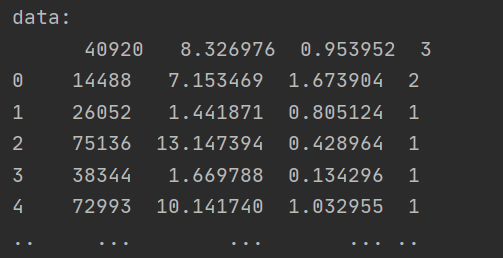3、标准化

from sklearn.preprocessing import StandardScaler

意义

归一化比较容易收到最大值最小值的影响，如果出现异常值，对结果影响较大，俗称鲁棒性较差,故提出标准化，即使出现异常值，也不会影响较大。

公式
X ′ = x − m e a n σ X' =\frac{x-mean}{\sigma}

作用于每一列，mean为平均值，σ为标准差

代码

from  sklearn.preprocessing import StandardScaler
def standarScaler_demo():
"""
数据标准化
:return:
"""
# 1 数据导入

# 2 实例化standscaler
transf = StandardScaler()

# 3 fit_transform()
data_new = transf.fit_transform(data)
print("data:\n ",data_new)

if __name__ == "__main__":
# 代码9 数据标准化
standarScaler_demo()

[外链图片转存失败,源站可能有防盗链机制,建议将图片保存下来直接上传(img-NmMHR2Ib-1637326542944)(https://i.loli.net/2021/11/07/B83tjmgeQApnbF5.png)]

4、特征降维

**降维是指在某些限定条件下，降低随机变量(特征)个数，得到一组“不相关”主变量的过程。（相关特征：两个有关系的特征，例如湿度与降雨量）

1、降维方式一–特征选择

（1）定义

数据中包含冗余或无关变量（或称特征、属性、指标等），旨在从原有特征中找出主要特征

（2）方法
• Filter(过滤式)：主要探究特征本身特点、特征与特征和目标值之间关联
• 方差选择法：低方差特征过滤
• 相关系数：特征与特征之间的相关程度
• Embedded (嵌入式)：算法自动选择特征（特征与目标值之间的关联）
• 决策树:信息熵、信息增益
• 正则化：L1、L2
• 深度学习：卷积等
（3）模块
from sklearn.feature_selection import VarianceThreshold

2、征选择方式一 过滤式

删除低方差的一些特征，前面讲过方差的意义。再结合方差的大小来考虑这个方式的角度。

• 特征方差小：某个特征大多样本的值比较相近
• 特征方差大：某个特征很多样本的值都有差别

API

• sklearn.feature_selection.VarianceThreshold(threshold = 0.0)
• 删除所有低方差特征
• Variance.fit_transform(X)
• X:numpy array格式的数据[n_samples,n_features]
• 返回值：训练集差异低于threshold的特征将被删除。默认值是保留所有非零方差特征，即删除所有样本中具有相同值的特征。
from sklearn.feature_selection import VarianceThreshold

def variance_demo():
"""
过滤式处理低方差
:return:
"""
# 1 导入数据集

# 2 实例化VarianceThreshold转换器
transfer = VarianceThreshold(threshold=11)
#threshold表示设置的阈值，我们可以把小于这个阈值的删除，即为删除相关性比较强的内容

# 3 调用 fit_transform
data_new = transfer.fit_transform(data.iloc[:,1:-2])
print("data\n",data_new)
print("形状\n",data_new.shape)
print("原来形状\n",data.shape)
return None

if __name__ == "__main__":
# 代码10 低方差过滤
variance_demo()

data
[[ 5.95720000e+00  8.52525509e+10  8.00800000e-01 ...  1.21144486e+12
2.07014010e+10  1.08825400e+10]
[ 7.02890000e+00  8.41133582e+10  1.64630000e+00 ...  3.00252062e+11
2.93083692e+10  2.37834769e+10]
[-2.62746100e+02  5.17045520e+08 -5.67800000e-01 ...  7.70517753e+08
1.16798290e+07  1.20300800e+07]
...
[ 3.95523000e+01  1.70243430e+10  3.34400000e+00 ...  2.42081699e+10
1.78908166e+10  1.74929478e+10]
[ 5.25408000e+01  3.28790988e+10  2.74440000e+00 ...  3.88380258e+10
6.46539204e+09  6.00900728e+09]
[ 1.42203000e+01  5.91108572e+10  2.03830000e+00 ...  2.02066110e+11
4.50987171e+10  4.13284212e+10]]
形状
(2318, 7)
原来形状
(2318, 12)

3、皮尔逊相关系数(Pearson Correlation Coefficient)

反映变量之间相关关系密切程度的统计指标

相关系数的值介于–1与+1之间，即–1≤ r ≤+1。其性质如下

• 当r>0时，表示两变量正相关，r<0时，两变量为负相关
• 当|r|=1时，表示两变量为完全相关，当r=0时，表示两变量间无相关关系
• 当0<|r|<1时，表示两变量存在一定程度的相关。且|r|越接近1，两变量间线性关系越密切；|r|越接近于0，表示两变量的线性相关越弱
• 一般可按三级划分：|r|<0.4为低度相关；0.4≤|r|<0.7为显著性相关；0.7≤|r|<1为高度线性相关

API

from scipy.stats import pearsonr

from scipy.stats import pearsonr
import matplotlib.pyplot as plt

def pearsonr_demo():
"""
相关系数计算
:return: None
"""

factor = ['pe_ratio', 'pb_ratio', 'market_cap', 'return_on_asset_net_profit', 'du_return_on_equity', 'ev',
'earnings_per_share', 'revenue', 'total_expense']

for i in range(len(factor)):
for j in range(i, len(factor) - 1):
print(
"指标%s与指标%s之间的相关性大小为%f" % (factor[i], factor[j + 1], pearsonr(data[factor[i]], data[factor[j + 1]])))

plt.figure(figsize=(20, 8), dpi=100)
plt.scatter(data['revenue'], data['total_expense'])
plt.show()
return None
if __name__ == "__main__":

# 代码11 计算皮尔逊相关系数
pearsonr_demo()

指标pe_ratio与指标pb_ratio之间的相关性大小为-0.004389
指标pe_ratio与指标market_cap之间的相关性大小为-0.068861
指标pe_ratio与指标return_on_asset_net_profit之间的相关性大小为-0.066009
指标pe_ratio与指标du_return_on_equity之间的相关性大小为-0.082364
指标pe_ratio与指标ev之间的相关性大小为-0.046159
指标pe_ratio与指标earnings_per_share之间的相关性大小为-0.072082
指标pe_ratio与指标revenue之间的相关性大小为-0.058693

[外链图片转存失败,源站可能有防盗链机制,建议将图片保存下来直接上传(img-KDoPiIxN-1637326542945)(https://i.loli.net/2021/11/07/lTZ67OE9t1pka4u.png)]

5、主成分分析

1、介绍

• 定义：高维数据转化为低维数据的过程，在此过程中可能会舍弃原有数据、创造新的变量
• 作用：是数据维数压缩，尽可能降低原数据的维数（复杂度），损失少量信息。
• 应用：回归分析或者聚类分析当中

2、apl

from sklearn.decomposition import PCA

sklearn.decomposition.PCA(n_components=None)

• n_components:
• 小数：表示保留百分之多少的信息
• 整数：减少到多少特征
• PCA.fit_transform(X) X:numpy array格式的数据[n_samples,n_features]
• 返回值：转换后指定维度的array
from sklearn.decomposition import PCA

def PCA_demo():
"""
主成分分析降维
:return:
"""
# 1 导入数据
data = [[1,2,3,4],[5,6,7,8],[9,10,11,12]]
# 2 实例化
transfer = PCA(n_components=0.5)
# 3 调用fit_funcition函数
data_new = transfer.fit_transform(data)
print("data_new\n",data_new)

return None

if __name__ == "__main__":
# 代码12 PCA降维
PCA_demo()

保留90%的信息，降维结果为：
[[ -3.13587302e-16   3.82970843e+00]
[ -5.74456265e+00  -1.91485422e+00]
[  5.74456265e+00  -1.91485422e+00]]
降维到3维的结果：
[[ -3.13587302e-16   3.82970843e+00   4.59544715e-16]
[ -5.74456265e+00  -1.91485422e+00   4.59544715e-16]
[  5.74456265e+00  -1.91485422e+00   4.59544715e-16]]

三、分类算法

1、sklearn转换去和估计器

1、转换器

特征工程父类，所有的特征工程都会调用这个

• 实例化（Transformer）
• 调用fit_transform()方法

2、估计器（estimaor）

sklearn机器学习算法的实现

• 实例化一个estimateor

• 生成模型

​ estimator.fit(x_train,y_train)

• 评估模型好坏
• 法一、直接对比真实值与预测值

y_predict = estimato.predict(x_test)

t_test == y_predict

• 法二、计算准确率

accuracy = estimator.score(x_test,y_test)

2、KNN算法)(K近邻算法)

1、原理:根据邻居推算类别

分类时，对新的实例，根据其 k 个最近邻的训练实例的类别，通过多数表决等方式进行预测。k 值的选择，距离度量和分类决策规则是 k 近邻算法的三个基本要素

[外链图片转存失败,源站可能有防盗链机制,建议将图片保存下来直接上传(img-Y1xPCPa0-1637326542946)(https://i.loli.net/2021/11/09/YebpDgyFwc7QzVW.png)]

2、距离度量

特征空间中两个实例点的距离时两个实例点相似程度的反映.

• 闵可夫斯基距离

[外链图片转存失败,源站可能有防盗链机制,建议将图片保存下来直接上传(img-eVzT7f93-1637326542948)(https://i.loli.net/2021/11/09/YDIk3cf1psqnUyG.png)]

• 欧氏距离：p=2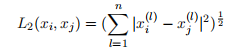• 曼哈顿距离：p=1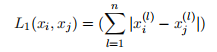• p=无穷：表示距离坐标最大值

[外链图片转存失败,源站可能有防盗链机制,建议将图片保存下来直接上传(img-muxYwXx8-1637326542951)(https://i.loli.net/2021/11/09/8XT2ZRKJHwUFb9r.png)]

3、k值的选择

给定一个训练数据集，对新的输入实例，在训练数据集中找到与该实例最邻近的k个实例，这k个实例的多数属于哪个类，就把该输入实例分为那个类

如果选择较小的 k 值，就相当于用较小的领域中的训练实例进行预测，只有与输入实例较近的的训练实例才会对预测结果起作用。但缺点是预测结果会对近邻的实例点非常敏感。这意味着整体模型变得更复杂，容易发生过拟合.

相反如果选择较大的 k 值，就相当于用较大领域中的训练实例进行预测. 这时与输入实例较远的训练实例也会对预测起作用，使预测发生错误。k 值的增大就意味着整体的模型变得简单。如果 k=N, 那么无论输入实例是什么，都将简单地预测它属于在训练实例中最多的类。这时，模型过于简单，完全忽略训练实例中的大量有用信息。

[外链图片转存失败,源站可能有防盗链机制,建议将图片保存下来直接上传(img-WAwQxWov-1637326542952)(https://i.loli.net/2021/11/09/OsIyqMTzC14nwxK.png)]

放入一个待分类的实例

• k = 1，按照某种距离度量找到最邻近的1个

训练样本测试实例的类别，判定为类1

• k = 3，按照某种距离度量找到最邻近的3个

训练样本测试实例的类别，判定为类2

• k = 5，按照某种距离度量找到最邻近的5个

训练样本测试实例的类别，判定为类1

4、KNN三要素

• K值选择
• 距离度量
• 分类决策规则（常采用投票规则）

5、APL

• #第1步：从sklearn中导入模型
from sklearn.neighbors import KNeighborsClassifier
sklearn.neighbors.KNeighborsClassifier(n_neighbors=5,algorithm='auto')

#第2步：创建模型的实例
knn=KNeighborsClassifier(n_neighbors=k,algorithm='auto')
# n_neighbors：int,可选（默认= 5），k_neighbors查询默认使用的邻居数,k值。
#algorithm：{‘auto’，‘ball_tree’，‘kd_tree’，‘brute’}，可选用于计算最近邻居的算法：‘ball_tree’将会使用 BallTree，‘kd_tree’将使用 KDTree。‘auto’将尝试根据传递给fit方法的值来决定最合适的算法。 (不同实现方式影响效率)
#p 计算距离的方式p=2表示欧氏距离
#第3步：模型训练
knn.fit(X_train,y_train)

#第4步：预测待分类数据的类别标签
y_pred=knn.predict(X_test)

#第1步：从sklearn中导入模型
from sklearn.neighbors import KNeighborsRegressor

# 第2步：创建模型的实例
knn = KNeighborsRegressor(n_neighbors=2

#第3步：模型训练
knn.fit(X_train,y_train)

# 第4步：预测待分类数据的类别标签
y_pred=knn.predict(X_test)

6、案例一：鸢尾花

from sklearn.neighbors import KNeighborsClassifier#KNN算法
from sklearn.model_selection import  train_test_split#数据划分
from  sklearn.preprocessing import  StandardScaler#标准化
# 鸢尾花种类分类
def knn_iris():
"""
用KNN对鸢尾花种类分类
:return:
"""
# (1) 获取数据

# （2）划分数据集
x_train,x_test,y_train,y_test = train_test_split(iris.data,iris.target,random_state=6)

# （3）特征工程：标准化
transfer = StandardScaler()
x_train= transfer.fit_transform(x_train)#训练集要标准化
x_test = transfer.fit_transform(x_test)#测试集也要标准化

# （4）KNN 算法预估器
knn = KNeighborsClassifier(n_neighbors=3)
knn.fit(x_train,y_train)

# （5）模型评估
# 法一：直接比对真实值和预测值
y_predict = knn.predict(x_test)
print("y_predice:\n",y_predict)
print("直接对比真实值和预测值:\n",y_test==y_predict)
# 法二：计算准确率
score = knn.score(x_test,y_test)
print("准确率为：\n",score)

return None

if __name__ == "__main__":
# 代码1 用KNN对鸢尾花尽心种类预测
knn_iris()

7、案例二、预测签到位置

import pandas as pd
import numpy as np
from sklearn.neighbors import KNeighborsClassifier #KNN
from sklearn.model_selection import train_test_split#数据集的划分
from sklearn.preprocessing import StandardScaler#标准化
from sklearn.model_selection import GridSearchCV#网格搜索

#数据处理
# 缩小数据集范围
data = data.query("x > 1 & x < 1.25 & y > 2.5 & y < 2.75")
data
# 将时间转换为周、日、小时
# print(data["time"])
time_value = pd.to_datetime(data['time'],unit='s')#将时间戳转换成日期格式，时间戳单位是秒
# print(time_value)
time_value = pd.DatetimeIndex(time_value)#转为datetimeindex数据格式以便于后续处理
# print(time_value)
data['day'] = time_value.day
data['hour']=time_value.hour
data['weekday']= time_value.weekday
pritn(data.describe)
# 删除没用的日期数据
data  = data.drop(['time'],axis = 1)
print(data)
# 将签到位置少于n个用户的删除
place_count = data.groupby("place_id").count()['row_id']#计算每一个地点签到的次数
print(place_count)
#用data中的ID与筛选出来的id进行匹配，显示筛选出的行
data_final = data[data['place_id'].isin(place_count[place_count > 3 ].index.values)]
print(data_final)
# 筛选特征值和目标值
X = data_final[['x','y','accuracy','hour','day','weekday']]
y = data_final['place_id']

#数据集划分
X_train,X_test,y_train,y_test = train_test_split(X,y,test_size=0.2)

# 标准化
transfer = StandardScaler()
X_train = transfer.fit_transform(X_train)
X_test = transfer.fit_transform(X_test)

# KNN算法预估器
estimator = KNeighborsClassifier()
# 网格搜索
param_dict = {"n_neighbors":[1,3,5,7,9]}
estimator = GridSearchCV(estimator,param_grid=param_dict,cv=3)
estimator.fit(X_train,y_train)

# 模型评估
# 方法1：
y_predict = estimator.predict(X_test)
print("真实值与预测值：\n",y_test == y_predict)
# 方法2:
score = estimator.score(X_test,y_test)
print("准确率：",score)

# 最佳参数
print("最佳参数:",estimator.best_params_)
print("最佳结果：",estimator.best_score_)
print("最佳估计器：",estimator.best_estimator_)
print("交叉验证结果：",estimator.cv_results_)

8、评价

• 优点：简单，易于理解，易于实现，无需训练
• 缺点：
• 懒惰算法，对测试样本分类时的计算量大，内存开销大
• 必须指定K值，K值选择不当则分类精度不能保证
• 使用场景：小数据场景，几千～几万样本，具体场景具体业务去测试

3、朴素贝叶斯算法（朴素即为相互独立+贝叶斯）

1、流程

• 找到特征值X={x1,x2,x3,…xm}(X={有星星，有云，有风…})
• 找到标签值y={y1,y2,…yn}（y={晴天、阴天}）
• 分别计算后验概率p(y1|X)、p(y2|X)、p(y3|X)…p(yn|X)
• 如果p(yk|X)=max{p(y1|X),p(y2|X)…p(yn|X)}则yk为最终预测值

2、概念

• 完备事件组：把全集切分成几个子集，这几个子集互不相容、称这些子集为完备事件组

• 条件概率：后验概率，表示实验中各种"原因"可能发生的大小

P（A|B）:在B发生条件下A发生的概率

• 相互独立：P（A，B ）= P( A ) * P( B )

• 先验概率：在测试之前是已知的，一半来自过往的经验

• 全概率：
P ( A ) = ∑ n = 1 x P ( B i ) P ( A ∣ B i ) P(A) = \displaystyle \sum^x_{n=1}P(B_i)P(A|B_i)
把每一部分A的概率加起来其中 A概率为B的概率*B发生条件下A发生概率，B为完备事件组

• 贝叶斯公式
P ( B k ∣ A ) = P ( B k ∣ A ) P ( A ) = P ( B k ) P ( A ∣ B k ) ∑ i = 1 n P ( B i ) P ( A ∣ B i ) , k = 1 , 2 , 3... , n P(B_k|A)=\frac{P(B_k|A)}{P(A)}=\frac{P(B_k)P(A|B_k)}{\displaystyle \sum^n_{i=1}P(B_i)P(A|B_i)},k=1,2,3...,n

• 朴素贝叶斯

假设特征与特征之间相互独立

3、例子

[外链图片转存失败,源站可能有防盗链机制,建议将图片保存下来直接上传(img-nNnfyupd-1637326542953)(https://i.loli.net/2021/11/16/wuWBXarEzShAJH5.png)]

• 女神喜欢的概率

P = 4 / 7 P=4/7

职业是程序员并且女神喜欢的概率
P = 1 / 7 P=1/7
在女神喜欢的条件下，职业是程序员概率
P = 2 / 4 P =2/4
在女神喜欢条件下，职业是程序员 体重超重的概率是多少？
P = 1 / 4 P= 1/4
小明是产品经理，超重，问女神喜欢的概率是多少？

分子：P(产品经理，超重|喜欢) * P（喜欢）

分母：P（产品经理，超重）
p(程序员, 匀称) =  P(程序员)P(匀称) =3/7*(4/7) = 12/49
P(产品, 超重|喜欢) = P(产品|喜欢)P(超重|喜欢)=1/2 *  1/4 = 1/8

4、拉普拉斯平滑系数

防止计算出的分类概率为0
P ( F 1 ∣ C ) = N i + α N + α m P(F_1|C)=\frac{N_i+\alpha}{N+{\alpha}m}

α 表 示 为 指 定 系 数 ， 一 般 为 1 ， m 表 示 训 练 文 档 总 统 计 出 的 特 征 词 个 数 。 {\alpha}表示为指定系数，一般为1，m表示训练文档总统计出的特征词个数。

5、apl

• sklearn.naive_bayes.MultinomialNB(alpha = 1.0)
• 朴素贝叶斯分类
• alpha：拉普拉斯平滑系数

6、代码

from sklearn.datasets import fetch_20newsgroups
from sklearn.model_selection import train_test_split
from sklearn.feature_extraction.text import TfidfVectorizer#文本特征提取
from sklearn.naive_bayes import MultinomialNB
def naive_bayes():
"""
朴素贝叶斯对新闻进行分类
:return:
"""
# 1获取数据
news = fetch_20newsgroups(data_home='data',subset="all")
# data_home:指定数据获取后下载到哪里
# subset:数据获取什么，默认获取train,想要获取所有数据要指定为all

# 2划分数据集
X_train,X_test,y_train,y_test = train_test_split(news.data,news.target,train_size=0.2,random_state=1)

# 3特征工程：文本特征抽取-tfidf
transfer = TfidfVectorizer()
X_train = transfer.fit_transform(X_train)
X_test = transfer.transform(X_test)

# 4朴素贝叶算法进行模型预估流程
estimator = MultinomialNB()
estimator.fit(X_train,y_train)

# 5模型评估
# 法一：直接比对真实值和预测值
y_predict = estimator.predict(X_test)
print("y_predice:\n", y_predict)
print("直接对比真实值和预测值:\n", y_test == y_predict)
# 法二：计算准确率
score = estimator.score(X_test, y_test)
print("准确率为：\n", score)
return

if __name__ == "__main__":
naive_bayes()

7、总结

• 优点：
• 朴素贝叶斯模型发源于古典数学理论，有稳定的分类效率。
• 对缺失数据不太敏感，算法也比较简单，常用于文本分类。
• 分类准确度高，速度快
• 缺点：
• 由于使用了样本属性独立性的假设，所以如果特征属性有关联时其效果不好

4、决策树(找到最高效的决策顺序)

1、公式+原理

（1）信息熵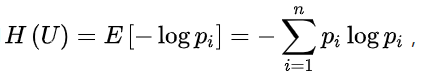（2）信息增益（ID3）

[外链图片转存失败,源站可能有防盗链机制,建议将图片保存下来直接上传(img-QH2xNR4k-1637326542955)(https://i.loli.net/2021/11/11/mDxhIle38PtZAky.png)]
信 息 增 益 = 信 息 熵 − 加 权 熵 信息增益 = 信息熵-加权熵
（3）信息增益率(C4.5)

对信息增益的改进，防止模型对样本数目过多偏爱。其中的HA(D)，对于样本集合D，将当前特征A作为随机变量（取值是特征A的各个特征值），求得的经验熵。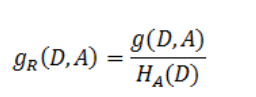是在信息增益的基础之上乘上一个惩罚参数。特征个数较多时，惩罚参数较小；特征个数较少时，惩罚参数较大是在信息增益的基础之上乘上一个惩罚参数。特征个数较多时，惩罚参数较小；特征个数较少时，惩罚参数较大

（4）gini系数(CART–二叉树)

只有二叉树可以处理连续数据，多类数据需要先看成二叉树在进行下一步划分。

Gini指数越小表示集合中被选中的样本被分错的概率越小，也就是说集合的纯度越高，反之，集合越不纯。

[外链图片转存失败,源站可能有防盗链机制,建议将图片保存下来直接上传(img-JL9wr1LF-1637326542958)(https://i.loli.net/2021/11/11/845VT27DLhOtvIo.png)]

​ 假设集合中有K个类别:

[外链图片转存失败,源站可能有防盗链机制,建议将图片保存下来直接上传(img-hnI2xTVE-1637326542960)(https://i.loli.net/2021/11/11/382xspqUOGn4bom.png)]

2、计算举例

已知四个特征，预测是否贷款给某人。

[外链图片转存失败,源站可能有防盗链机制,建议将图片保存下来直接上传(img-zRkS67iu-1637326542961)(https://i.loli.net/2021/11/16/H49oL627Dk5izGO.png)]

信息熵：
H ( 总 ) = ( 6 15 ∗ l o g 2 9 15 + 9 15 ∗ l o g 2 6 15 ) ≈ 0.971 H(总)=(\frac{6}{15}*log_2{\frac{9}{15}} + \frac{9}{15}*log_2{\frac{6}{15}})\approx0.971
信息增益：
g ( D , 年 龄 ) = H ( 总 ) − H ( D ∣ 年 龄 ) g(D,年龄)=H(总)-H(D|年龄)

H ( D ∣ 年 龄 ) = 5 15 ∗ H ( 青 年 ) + 5 15 ∗ H ( 中 年 ) + 5 15 ∗ H ( 老 年 ) H(D|年龄)=\frac{5}{15}*H(青年)+\frac{5}{15}*H(中年)+\frac{5}{15}*H(老年)

H ( 青 年 ) = − ( 2 5 ∗ l o g 2 2 5 + 3 5 ∗ l o g 2 3 5 ) H(青年)= -(\frac{2}{5}*log_2\frac {2}{5} + \frac{3}{5}*log_2\frac{3}{5})

H ( 中 年 ) = − ( 2 5 ∗ l o g 2 2 5 + 3 5 ∗ l o g 2 3 5 ) H(中年)= -(\frac{2}{5}*log_2\frac {2}{5} + \frac{3}{5}*log_2\frac{3}{5})

H ( 老 年 ) = − ( 1 5 ∗ l o g 2 1 5 + 4 5 ∗ l o g 2 4 5 ) H(老年)= -(\frac{1}{5}*log_2\frac {1}{5} + \frac{4}{5}*log_2\frac{4}{5})

g(D, A1)g(D, A2)g(D, A3) maxg(D, A4)
0.3130.3240.4200.363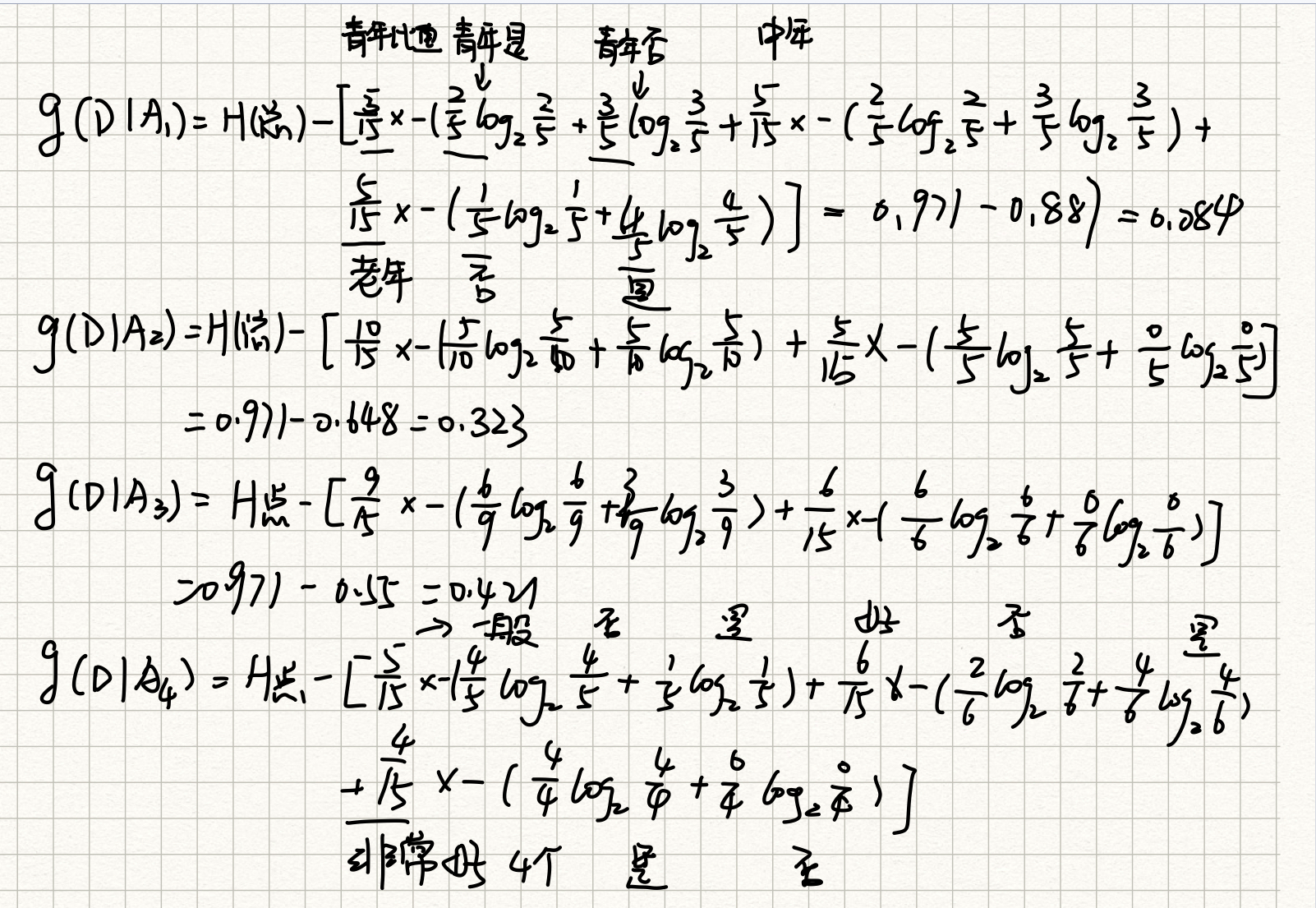GINI: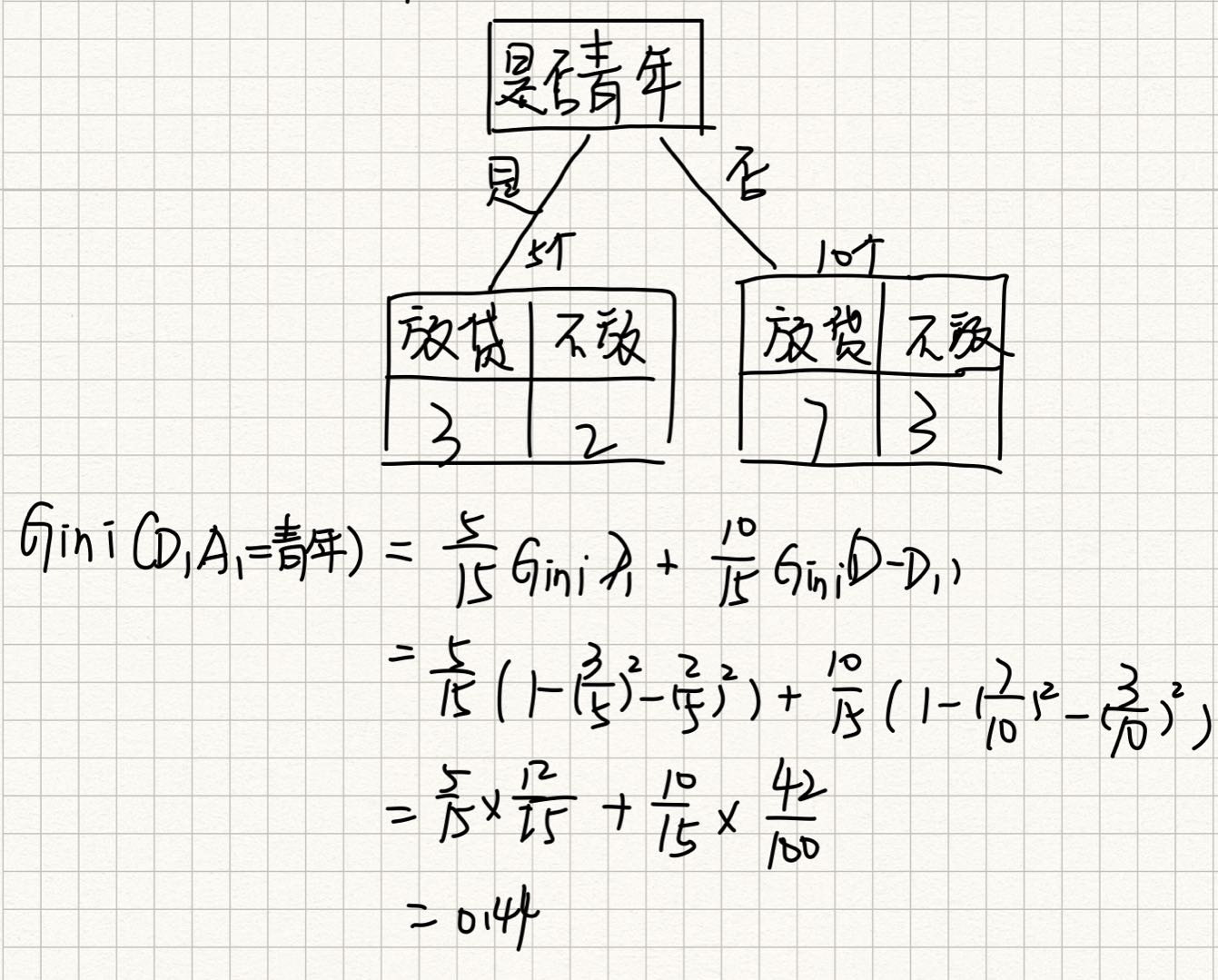3、分类

• ID3：信息增益 最大的准则

存在偏向于选择特征值较多的特征

• C4.5：信息增益比 最大的准则

信息增益比偏向取值较少的特征

基于以上缺点，并不是直接选择信息增益率最大的特征，而是现在候选特征中找出信息增益高于平均水平的特征，然后在这些特征中再选择信息增益率最高的特征

• CART

• 分类树: 基尼系数 最小的准则 在sklearn中可以选择划分的默认原则
• 优势：划分更加细致（从后面例子的树显示来理解）

4、APL

class sklearn.tree.DecisionTreeClassifier(criterion=’gini’, max_depth=None,random_state=None)

• 决策树分类器
• criterion:默认是’gini’系数，也可以选择信息增益的熵’entropy’
• max_depth:树的深度大小
• random_state:随机数种子

5、代码

from sklearn.model_selection import train_test_split
from sklearn.tree import DecisionTreeClassifier
def tree_demo():
"""
使用决策树对鸢尾花进行分类处理
:return:
"""
# 获取数据集

# 划分数据集
X_train,X_test,y_train,y_test = train_test_split(data.data,data.target,random_state=11,train_size=0.8)

# 决策树特征工程
estimator =DecisionTreeClassifier()
estimator.fit(X_train,y_train)

# 模型评估
y_pre = estimator.predict(X_test)
print("预测值",y_pre)

score = estimator.score(X_test,y_test)
print("准确率",score)
return None

if __name__ == "__main__":
tree_demo()

6、将树的结构导出

方式1：

import graphviz
import tree

dot_data = tree.export_graphviz(estimator, out_file=None,
class_names=["琴酒", '伏特加', '雪莉'],  # 中文会出现乱码
filled=True,  # 是否填充颜色
rounded=True,  # 框的形状
special_characters=True)
graph = graphviz.Source(dot_data.replace('helvetica', '"Microsoft YaHei"'), encoding='utf-8')
graph.render('data/tree_iris')  # 生成一个pdf到指定文件夹下
graph = pydotplus.graph_from_dot_data(dot_data)
Image(graph.create_png())  # 显示代码,pycharm显示不出来，jupyter可以

方式2：

from sklearn.tree import export_graphviz

tree.export_graphviz(estimator,out_file="data\iris_tree.dot")
#生成是一个dox文件，需要在网站上解析

7、泰坦尼克号

from sklearn.model_selection import train_test_split
from sklearn.tree import export_graphviz
from sklearn.tree import DecisionTreeClassifier
import graphviz
import pandas as pd
##根据构建的决策树模型画出决策图像
# 这里需要安装pydotplus库以及graphviz这个软件，才能输出下面的图
# 可以参照：https://blog.csdn.net/qq_40304090/article/details/88594813
from sklearn import tree
import pydotplus
from IPython.display import Image
from sklearn.feature_extraction import  DictVectorizer

def Titanic_mode():
"""
决策树处理泰坦尼克
:return:
"""
# 获取数据

# 选择特征以及标签
x = data[['Pclass','Sex','Age']]
y = data['Survived']

# 数据处理
#缺失值
x['Age'].fillna(x['Age'].mean(),inplace=True)

#特征值转为字典类
dict = DictVectorizer(sparse=False)
x = dict.fit_transform(x.to_dict(orient='records'))
print(dict.get_feature_names())

# 划分数据集
X_train,X_test,y_train,y_test = train_test_split(x,y,random_state=11,train_size=0.8)

# 字典特征抽取

# 决策树
# 决策树特征工程
estimator = DecisionTreeClassifier()
estimator.fit(X_train, y_train)

# 模型评估
y_pre = estimator.predict(X_test)
print("预测值", y_pre)

score = estimator.score(X_test, y_test)
print("准确率", score)

# 可视化决策树
# 方式1
# tree.export_graphviz(estimator, out_file="data\iris_tree.dot")
# 方式2

dot_data = tree.export_graphviz(estimator, out_file=None,
# 中文会出现乱码
filled=True,  # 是否填充颜色
rounded=True,  # 框的形状
special_characters=True)
graph = graphviz.Source(dot_data.replace('helvetica', '"Microsoft YaHei"'), encoding='utf-8')  # 中文乱码问题
graph.render('data/tree_Titanic')  # 生成一个pdf到指定文件夹下
graph = pydotplus.graph_from_dot_data(dot_data)
Image(graph.create_png())  # 显示代码,pycharm显示不出来，jupyter可以
return None

if __name__ == "__main__":
# tree_demo()
Titanic_mode()

5、随机森林

1、集成学习方法

集成学习通过建立几个模型组合的来解决单一预测问题。它的工作原理是生成多个分类器/模型，各自独立地学习和作出预测。这些预测最后结合成组合预测，因此优于任何一个单分类的做出预测。

2、随机森林方法

• 训练集随机：随机有放回抽样，生成训练集
• 特征随机：从M个特征中随机抽取m个特征（M>>m）可以实现降维
• [外链图片转存失败,源站可能有防盗链机制,建议将图片保存下来直接上传(img-cD9oqZDK-1637326542962)(https://i.loli.net/2021/11/16/YbBzXsgVNAt7Dme.png)]

3、APL

• class sklearn.ensemble.RandomForestClassifier(

n_estimators=10, criterion=’gini’, max_depth=None, bootstrap=True, random_state=None, min_samples_split=2)

• 随机森林分类器
• n_estimators：integer，optional（default = 10）森林里的树木数量120,200,300,500,800,1200
• criteria：string，可选（default =“gini”）分割特征的测量方法
• max_depth：integer或None，可选（默认=无）树的最大深度 5,8,15,25,30
• max_features="auto”,每个决策树的最大特征数量
• If “auto”, then max_features=sqrt(n_features).
• If “sqrt”, then max_features=sqrt(n_features) (same as “auto”).
• If “log2”, then max_features=log2(n_features).
• If None, then max_features=n_features.
• bootstrap：boolean，optional（default = True）是否在构建树时使用放回抽样
• min_samples_split:节点划分最少样本数
• min_samples_leaf:叶子节点的最小样本数
• 超参数：n_estimator, max_depth, min_samples_split,min_samples_leaf

4、鸢尾花评估

from sklearn.ensemble import RandomForestClassifier
from sklearn.model_selection import train_test_split
import pandas as pd
from sklearn.feature_extraction import  DictVectorizer
from sklearn.model_selection import GridSearchCV#网格搜索

def random_foreat():
"""
使用随机森林对泰坦尼克号进行预测
:return:
"""

# 选择特征以及标签
x = data[['Pclass', 'Sex', 'Age']]
y = data['Survived']

# 数据处理
# 缺失值
x['Age'].fillna(x['Age'].mean(), inplace=True)

# 特征值转为字典类
dict = DictVectorizer(sparse=False)
x = dict.fit_transform(x.to_dict(orient='records'))
print(dict.get_feature_names())

# 划分数据集
X_train, X_test, y_train, y_test = train_test_split(x, y, random_state=11, train_size=0.8)

#随机森林模型训练
estimator = RandomForestClassifier()
# 网格搜索
param_dict = {"n_estimators": [120,200,300,500,800,1200], "max_depth": [5, 8, 15, 25, 30]}
estimator = GridSearchCV(estimator,param_grid=param_dict,cv=3)
estimator.fit(X_train,y_train)
y_pre = estimator.predict(X_test)
print("预测值", y_pre)

score = estimator.score(X_test, y_test)
print("准确率", score)

# 最佳参数
print("最佳参数:", estimator.best_params_)
print("最佳结果：", estimator.best_score_)
print("最佳估计器：", estimator.best_estimator_)
print("交叉验证结果：", estimator.cv_results_)
return None

if __name__ =="__main__":
random_foreat()

6、模型选择与评优

(1)交叉验证Cross Validation

交叉验证：此概念针对训练集，将拿到的训练数据，分为训练和验证集。以下图为例：将数据分成5份，其中一份作为验证集。然后经过5次(组)的测试，每次都更换不同的验证集。即得到5组模型的结果，取平均值作为最终结果。又称5折交叉验证。

将训练集训练好的模型利用test进行模型评估

[外链图片转存失败,源站可能有防盗链机制,建议将图片保存下来直接上传(img-3PLQGsaY-1637326542963)(https://i.loli.net/2021/11/09/qYNWkmRE1XvzBAb.png)]

[外链图片转存失败,源站可能有防盗链机制,建议将图片保存下来直接上传(img-VeUIuBw2-1637326542963)(https://i.loli.net/2021/11/09/WDPUo9phgRvkljB.png)]

（2）网格搜索Grid Search

每组超参数都采用交叉验证来进行评估。最后选出最优参数组合建立模型。

APL

sklearn.model_selection.GridSearchCV(knn, param_grid=None,cv=None)

• knn：估计器对象（KNN实力化了的模型）

• param_grid：估计器参数需要传入一个字典(dict){“n_neighbors”:[1,3,5]}

• cv：指定几折交叉验证

• fit：输入训练数据

• score：准确率

结果分析：

• print("最佳估计器： ",knn.best_estimator_)
print("最好结果 ",knn.best_score_)
print("每次交叉验证之后的准确率: ",knn.cv_results_)
print("最佳k: ", knn.best_params_)

from sklearn.neighbors import KNeighborsClassifier#KNN算法
from sklearn.model_selection import  train_test_split#数据划分
from  sklearn.preprocessing import  StandardScaler#标准化
from sklearn.model_selection import  GridSearchCV
def Titanic_mode():
"""
决策树处理泰坦尼克
:return:
"""
# 获取数据

# 选择特征以及标签
x = data[['Pclass','Sex','Age']]
y = data['Survived']

# 数据处理
#缺失值
x['Age'].fillna(x['Age'].mean(),inplace=True)

#特征值转为字典类
dict = DictVectorizer(sparse=False)
x = dict.fit_transform(x.to_dict(orient='records'))
print(dict.get_feature_names())

# 划分数据集
X_train,X_test,y_train,y_test = train_test_split(x,y,random_state=11,train_size=0.8)

# 字典特征抽取

# 决策树
# 决策树特征工程
estimator = DecisionTreeClassifier()
estimator.fit(X_train, y_train)

# 模型评估
y_pre = estimator.predict(X_test)
print("预测值", y_pre)

score = estimator.score(X_test, y_test)
print("准确率", score)

# 可视化决策树
# 方式1
# tree.export_graphviz(estimator, out_file="data\iris_tree.dot")
# 方式2

dot_data = tree.export_graphviz(estimator, out_file=None,
# 中文会出现乱码
filled=True,  # 是否填充颜色
rounded=True,  # 框的形状
special_characters=True)
graph = graphviz.Source(dot_data.replace('helvetica', '"Microsoft YaHei"'), encoding='utf-8')  # 中文乱码问题
graph.render('data/tree_Titanic')  # 生成一个pdf到指定文件夹下
graph = pydotplus.graph_from_dot_data(dot_data)
Image(graph.create_png())  # 显示代码,pycharm显示不出来，jupyter可以
return None

if __name__ == "__main__":
# 代码2 使用网格搜索找k值KNN鸢尾花进行分类
Grid_KNN_iris()

[外链图片转存失败,源站可能有防盗链机制,建议将图片保存下来直接上传(img-7jEkCJLi-1637326542964)(https://i.loli.net/2021/11/09/6y8UvBS2Nj4udkm.png)]

四、回归问题

1、线性回归

1、原理

线性回归(Linear regression)是利用回归方程(函数)对一个或多个自变量(特征值)和因变量(目标值)之间关系进行建模的一种分析方式。

自变量是一次称为单变量回归，大于一个自变量情况的叫做多元回归。

2、线性回归性能评估

法一：最小二乘

J ( θ ) = ( h w ( x 1 ) − y 1 ) 2 + ( h w ( x 2 ) − y 2 ) 2 + . . . + ( h w ( x m ) − y m ) 2 J(\theta)=(h_w(x_1)-y_1)^2+(h_w(x_2)-y_2)^2+...+(h_w(x_m)-y_m)^2

• y_i为第i个训练样本的真实值
• h(x_i)为第i个训练样本特征值组合预测函数
• 又称最小二乘法

法二：均方误差

M S E = 1 m ∑ i = 1 m ( y i − y ˉ ) 2 MSE = \frac{1}{m}\displaystyle \sum_{i=1}^m(y^i-\bar{y})^2
注：y^i为预测值，¯y为真实值

• sklearn.metrics.mean_squared_error(y_true, y_pred)
• 均方误差回归损失
• y_true:真实值
• y_pred:预测值
• return:浮点数结果

3、优化

方法一：梯度下降

w 1 = w 1 − α ∂ c o n t ( w 0 + w 1 x 1 ) ∂ w 1 w_1 = w_1-{\alpha}\frac{∂cont(w_0+w_1x_1)}{∂w_1}

w 0 = w 0 − α ∂ c o n t ( w 0 + w 1 x 1 ) ∂ w 1 w_0 = w_0-{\alpha}\frac{∂cont(w_0+w_1x_1)}{∂w_1}

α为学习速率，需要手动指定（超参数），α旁边的整体表示方向

沿着这个函数下降的方向找，最后就能找到山谷的最低点，然后更新W值

使用：面对训练数据规模十分庞大的任务 ，能够找到较好的结果

[外链图片转存失败,源站可能有防盗链机制,建议将图片保存下来直接上传(img-q4ykhlKx-1637326542965)(C:\Users\lenovo\AppData\Roaming\Typora\typora-user-images\image-20211117215140410.png)]

[外链图片转存失败,源站可能有防盗链机制,建议将图片保存下来直接上传(img-s8cafRId-1637326542965)(C:\Users\lenovo\AppData\Roaming\Typora\typora-user-images\image-20211117215152125.png)]

方法二：正规方程

w = ( X T X ) − 1 X T y w=(X^TX)^{-1}X^Ty

X为特征值矩阵，y为目标值矩阵。直接求到最好的结果

[外链图片转存失败,源站可能有防盗链机制,建议将图片保存下来直接上传(img-28OARJxj-1637326542966)(https://i.loli.net/2021/11/16/dz1vJrx9TcVisXy.gif)]

3、APL

• sklearn.linear_model.LinearRegression(fit_intercept=True)

• 通过正规方程优化
• fit_intercept：是否计算偏置
• LinearRegression.coef_：回归系数
• LinearRegression.intercept_：偏置
• sklearn.linear_model.SGDRegressor(loss=“squared_loss”, fit_intercept=True, learning_rate =‘invscaling’, eta0=0.01)

• SGDRegressor类实现了随机梯度下降学习，它支持不同的loss函数和正则化惩罚项来拟合线性回归模型。
• loss:损失类型
• loss=”squared_loss”: 普通最小二乘法
• fit_intercept：是否计算偏置
• learning_rate : string, optional
• 学习率填充
• ’constant’: eta = eta0
• ’optimal’: eta = 1.0 / (alpha * (t + t0)) [default]
• ‘invscaling’: eta = eta0 / pow(t, power_t)
• power_t=0.25:存在父类当中
• 对于一个常数值的学习率来说，可以使用learning_rate=’constant’ ，并使用eta0来指定学习率。
• SGDRegressor.coef_：回归系数
• SGDRegressor.intercept_：偏置

4、代码

使用正规方程+梯度下降对波士顿房价进行预测

from sklearn.model_selection import train_test_split
from sklearn.preprocessing import StandardScaler
from sklearn.linear_model import LinearRegression#正规方程
from sklearn.linear_model import  SGDRegressor#题梯度下降
from sklearn.metrics import mean_squared_error#均方误差
def liner_1():
"""
正规方程预测房屋价格

:return:
"""
# 1.数据获取

# 2.划分数据集
x_train , x_test , y_train, y_test = train_test_split(boston.data , boston.target , random_state=22)
# 3.标准化
transfer = StandardScaler()
x_train= transfer.fit_transform(x_train)
x_test  = transfer.transform(x_test)
# 4.预估器
estimator = LinearRegression()
estimator.fit(x_train,y_train)
# 5.得出模型
print("正规方程的权重系数,\n",estimator.coef_)
print("正规方程偏置：\n",estimator.intercept_)
# 6.模型评估
y_pre = estimator.predict(x_test)
print("房价预测：\n",y_pre)
erro = mean_squared_error(y_test,y_pre)
print("正规方程均方误差:\n",erro)
return None

def liner_2():
"""
梯度下降预测房屋价格

:return:
"""
# 1.数据获取

# 2.划分数据集
x_train , x_test , y_train , y_test =  train_test_split(boston.data , boston.target , random_state=22)
# 3.标准化
transfer = StandardScaler()
x_train= transfer.fit_transform(x_train)
x_test  = transfer.transform(x_test)
# 4.预估器
estimator =SGDRegressor(learning_rate='constant',#选择学习率
eta0=0.001,
max_iter=10000#设置迭代次数
)

estimator.fit(x_train,y_train)
# 5.得出模型
print("梯度下降的权重系数,\n",estimator.coef_)
print("梯度下降偏置：\n",estimator.intercept_)
# 6.模型评估
y_pre = estimator.predict(x_test)
print("房价预测：\n",y_pre)
erro = mean_squared_error(y_test,y_pre)
print("梯度下降均方误差:\n",erro)
return None

if __name__=="__main__":

liner_1()
liner_2()

梯度下降正规方程
需要选择学习率不需要
需要迭代求解一次运算得出
特征数量较大可以使用需要计算方程，时间复杂度高O(n3)
• 线性回归的损失函数-均方误差
• 线性回归的优化方法
• 正规方程
• 梯度下降
• 线性回归的性能衡量方法-均方误差
• sklearn的SGDRegressor API 参数

2、过拟合与欠拟合

[外链图片转存失败,源站可能有防盗链机制,建议将图片保存下来直接上传(img-5eMYWYHY-1637326542967)(https://i.loli.net/2021/11/18/s3ozfjTJNAa951V.png)]

1、过拟合问题解决方法

• L2正则化
• 作用：可以使得其中一些W的都很小，都接近于0，削弱某个特征的影响
• 优点：越小的参数说明模型越简单，越简单的模型则越不容易产生过拟合现象
• Ridge回归（岭回归）
• L1正则化
• 作用：可以使得其中一些W的值直接为0，删除这个特征的影响
• LASSO回归

2、岭回归

• sklearn.linear_model.Ridge(alpha=1.0, fit_intercept=True,solver=“auto”, normalize=False)
• 具有l2正则化的线性回归
• alpha:正则化力度（惩罚项系数），也叫 λ
• λ取值：0~1 1~10
• fit_intercept：是否添加偏置，True添加后更准确
• solver:会根据数据自动选择优化方法
• sag:如果数据集、特征都比较大，选择该随机梯度下降优化
• normalize:数据是否进行标准化
• normalize=False:可以在fit之前调用
• normalize=True 不用做标准化了
• preprocessing.StandardScaler标准化数据
• Ridge.coef_:回归权重
• Ridge.intercept_:回归偏置

Ridge方法相当于SGDRegressor(penalty=‘l2’, loss=“squared_loss”)

只不过SGDRegressor实现了一个普通的随机梯度下降学习，推荐使用Ridge(实现了SAG高级梯度下降)

def liner_3():
"""
岭回归 预测房屋价格

:return:
"""
# 1.数据获取

# 2.划分数据集
x_train , x_test , y_train, y_test = train_test_split(boston.data , boston.target , random_state=22)
# 3.标准化
transfer = StandardScaler()
x_train= transfer.fit_transform(x_train)
x_test  = transfer.transform(x_test)
# 4.预估器
estimator = Ridge()
estimator.fit(x_train,y_train)
# 5.得出模型
print("岭回归权重系数,\n",estimator.coef_)
print("岭回归偏置：\n",estimator.intercept_)
# 6.模型评估
y_pre = estimator.predict(x_test)
print("房价预测：\n",y_pre)
erro = mean_squared_error(y_test,y_pre)
print("岭回归均方误差:\n",erro)
return None

if __name__=="__main__":
# 岭回归
liner_3()

3、逻辑回归

1、了解逻辑回归

• 解决二分类问题的利器
• 线性回归的输出就是逻辑回归的输入
• 带入sigmoid函数中，输出结果【0，1】之间的概率值，默认0.5为阈值

2、sigmoid函数

g ( θ T x ) = 1 1 + e θ T x g(\theta^Tx)=\frac{1}{1+e^{\theta^Tx}}

[外链图片转存失败,源站可能有防盗链机制,建议将图片保存下来直接上传(img-ZBDr5VfJ-1637326542967)(https://i.loli.net/2021/11/18/ULjV1YdSfryGlBK.png)]

推导过程：

Logistic 回归模型是建立p/(1-p)与自变量之间关系的线性回归模型。用数学语言来表示，可以表达为：
l n p 1 − p = β 0 + β 1 x 1 + β 2 x 2 + . . . + β p x p + ϵ ln\frac{p}{1-p}=\beta_0+\beta_1x_1+\beta_2x_2+...+\beta_px_p+\epsilon

记 g ( x ) = β 0 + β 1 x 1 + β 2 x 2 + . . . + β p x p + ϵ , 得 到 记g(x) =\beta_0+\beta_1x_1+\beta_2x_2+...+\beta_px_p+\epsilon,得到

p = P ( y = 1 ∣ X ) = 1 1 + e − g ( x ) p = P(y=1|X)=\frac{1}{1+e^{-g(x)}}

p = P ( y = 0 ∣ X ) = 1 1 + e g ( x ) p = P(y=0|X)=\frac{1}{1+e^{g(x)}}

3、建模步骤

1、**分析模型：**根据分析目的设置指标变量（因变量与自变量），然后收集数据。

2、**模型估计：*y 取 1 的概率是 p = P(y = 1|*X), 取 0 的概率是 1 -p。用 ln (p/(1-p))和自变量列出线性回归方程，估计出模型中的回归系数。

3、**进行回归系数的显著性检验：**在多元线性回归中，回归方程显著并不意味着每个自变量对 y 的影响都是显著的，为了从回归方程中剔除那些次要的、可有可无的变量，重新建立更为简单有效的回归方程，需要对每个自变量进行显著性检验，检验结果由参数估计表得到。或采用逐步回归法，首先剔除掉最不显著的自变量，重新构造回归方程，一直到模型和参与的回归系数都通过检验。

4、**模型应用：**输入自变量的取值，就可以得到预测变量的值，或者根据预测变量的值取控

制自变量的取值

4、逻辑函数的损失

对数似然损失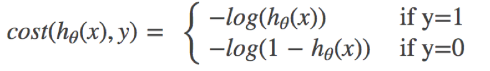C O S T ( h θ ( x ) , y ) = ∑ i = 1 m − y i l o g ( h θ ( x ) ) − ( 1 − y i ) l o g ( 1 − h θ ( x ) ) COST(h_\theta(x),y) = \sum^m_{i=1}-y_ilog(h_\theta(x))-(1-y_i)log(1-h_\theta(x))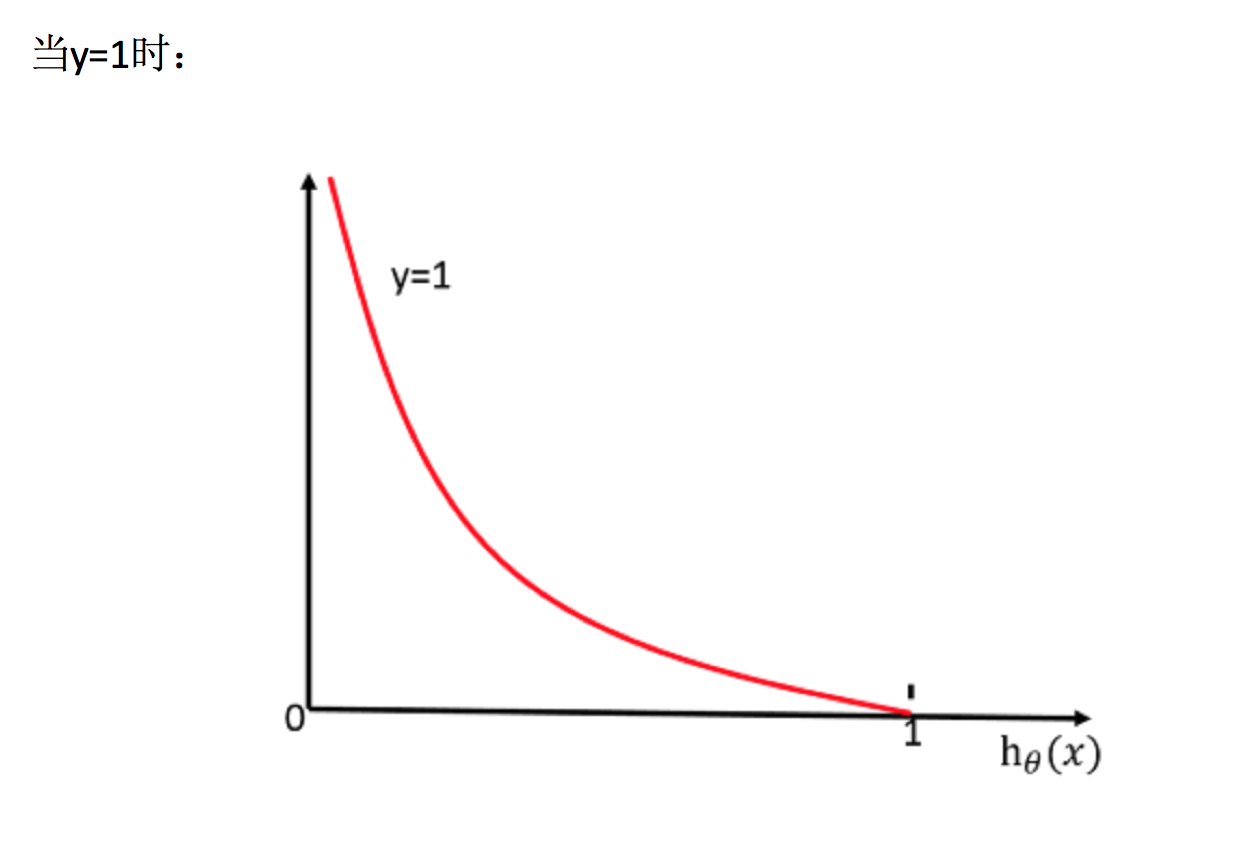[外链图片转存失败,源站可能有防盗链机制,建议将图片保存下来直接上传(img-Kk857HGD-1637326542968)(E:/机器学习/机器学习资料（黑马）/机器学习3天HTML/images/损失计算过程.png)]

5、APL

• sklearn.linear_model.LogisticRegression(solver=‘liblinear’, penalty=‘l2’, C = 1.0)
• solver:优化求解方式（默认开源的liblinear库实现，内部使用了坐标轴下降法来迭代优化损失函数）
• sag：根据数据集自动选择，随机平均梯度下降
• penalty：正则化的种类
• C：正则化力度

LogisticRegression方法相当于 SGDClassifier(loss=“log”, penalty=" ")

SGDClassifier实现了一个普通的随机梯度下降学习，也支持平均随机梯度下降法（ASGD），可以通过设置average=True。而使用LogisticRegression(实现了SAG)

6、肿瘤性质判断案例

from sklearn.preprocessing import StandardScaler
from sklearn.model_selection import train_test_split
from sklearn.linear_model import LogisticRegression
import numpy as np
import pandas as pd

def logistic_demo():
"""
通过逻辑回归对肿瘤性质进行预测
:return:
"""
# 获取数据（读取时加上names）
cloumn_names = ['Sample code number','Clump Thickness','Uniformity of Cell Size','Uniformity of Cell Shape','Marginal Adhesion',
'Single Epithelial Cell Size','Bare Nuclei','Bland Chromatin','Normal Nucleoli','Mitoses','Class']

# 数据处理(缺失值处理）
# 替换为np.nan
data = data.replace(to_replace='?',value=np.nan)
# 删除nan所在的行
data.dropna(inplace = True)
# 查看是否有缺失值
# print(data.isnull().any())

# 数据集划分
x = data.iloc[:,1:-1]
y = data.Class
X_train,X_test,y_train,y_test = train_test_split(x,y,random_state=11,train_size=0.7)

# 特征工程（无量纲）
transfer = StandardScaler()
X_train = transfer.fit_transform(X_train)
X_test = transfer.transform(X_test)

# 逻辑回归模型
estimator = LogisticRegression()
estimator.fit(X_train,y_train)
# 查看模型偏置
print("回归系数",estimator.coef_)
print("偏置",estimator.intercept_)

# 模型评估
y_pre = estimator.predict(X_test)
print("预测类别：\n",y_pre,y_test)
score = estimator.score(X_test,y_test)
print("准确率\n",score)
return None
if __name__=="__main__":

# 逻辑回归模型
logistic_demo()

4、精确率与召回率

1、混淆矩阵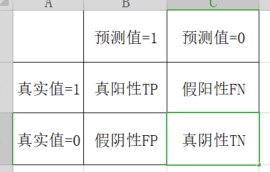• 准确率：预测值与真实值相符合的概率
T P + T N T P + F X + F P + T V \frac{TP+TN}{TP+FX+FP+TV}

• precision精确率：预测结果为真中，真实为正真的比例
T P T P + F P \frac{TP}{TP+FP}

• recall召回率：真实为真的样本中预测结果为真的比例（查的全，对正样本的区分能力）

T P T P + F N \frac{TP}{TP+FN}

• F!-score :反映了模型的稳健型
F 1 = 2 T P 2 T P + F N + F P = 2 ∗ P r e c i s i o n ∗ R e c a l l P r e c i s i o n + R e c a l l F1 = \frac{2TP}{2TP+FN+FP}=\frac{2*Precision*Recall}{Precision+Recall}

2、APL

sklearn.metrics.classification_report(y_true, y_pred, labels=[], target_names=None )

• y_true：真实目标值
• y_pred：估计器预测目标值
• labels:指定类别对应的数字，传类别是传的数字
• target_names：目标类别名称（要把类别的数字对应到具体的名字）
• return：每个类别精确率与召回率

[外链图片转存失败,源站可能有防盗链机制,建议将图片保存下来直接上传(img-5HWN2OA0-1637326542970)(https://i.loli.net/2021/11/19/dbm65kYQuwrayBl.png)]

3、ROC曲线+AUC指标

(1)应用

样本不均衡：样本正例反例数量差别过大

(2)原理

AUC 是ROC曲线与x围成的面积，越接近1越好，接近0.5越不好

• TPR = TP / (TP + FN)
• 所有真实类别为1的样本中，预测类别为1的比例
• FPR = FP / (FP + TN)
• 所有真实类别为0的样本中，预测类别为1的比例

[外链图片转存失败,源站可能有防盗链机制,建议将图片保存下来直接上传(img-KjgFxHTi-1637326542970)(https://i.loli.net/2021/11/19/GnpiXmdNsbjHqWT.png)]

• ROC曲线的横轴就是FPRate，纵轴就是TPRate，当二者相等时，表示的意义则是：对于不论真实类别是1还是0的样本，分类器预测为1的概率是相等的，此时AUC为0.5

（总共就两类，随便猜正确率最低为都猜同一种结果，效果最差为0.5，为了不让模型敷衍，有了AUC）

(3)APL

sklearn.metrics.roc_auc_score(y_true, y_pre)

• 计算ROC曲线面积，即AUC值
• y_true:每个样本的真实类别，必须为0(反例),1(正例)标记
• y_pre:每个样本预测的概率值

(4)ROC总结

• AUC只能用来评价二分类
• AUC非常适合评价样本不平衡中的分类器性能

5、K_means

处理没有标签的数据，进行聚类。属于无监督学习算法算法

1、原理

• 1、随机设置K个特征空间内的点作为初始的聚类中心（也可以手动设置）

• 2、对于其他每个点计算到K个中心的距离，未知的点选择最近的一个聚类中心点作为标记类别

• 3、接着对着标记的聚类中心之后，重新计算出每个聚类的新中心点（平均值）

• 4、如果计算得出的新中心点与原中心点一样（或者达到设置的计算次数），那么结束

[外链图片转存失败,源站可能有防盗链机制,建议将图片保存下来直接上传(img-pZ0NVmkb-1637326542971)(https://i.loli.net/2021/11/19/NDbwrQmHi8M53hV.png)]

中心点计算方法：

A（a1,b1,c1）
B (a2,b2,c2)
… … … …
Z(a26,b26,c26)
中心点 = （a平均，b平均，c平均）

2、k值选择方法

• 每次聚类完成后计算每个点到其所属的簇中心的距离的平方和，此平方和是会逐渐变小的，直到𝑘 = 𝑁时，平方和为0，因为每个点都是它所在的簇中心本身。但是在这个平方和变化过程中，会出现一个拐点也即“肘”，根据拐点确定K值。

[外链图片转存失败,源站可能有防盗链机制,建议将图片保存下来直接上传(img-Ni7bt7JO-1637326542972)(https://i.loli.net/2021/11/19/vyF8unIap4iZtxQ.png)]

3、APL

• sklearn.cluster.KMeans(n_clusters=8,init=‘k-means++’)
• k-means聚类
• n_clusters:开始的聚类中心数量（也就是K值）
• init:初始化方法，默认为’k-means ++’
• labels_:默认标记的类型，可以和真实值比较（不是值比较）

4、对Instacart Market用户进行聚类

from sklearn.cluster import KMeans
import pandas as pd
import numpy as np
from sklearn.preprocessing import StandardScaler
from sklearn.metrics import silhouette_score#轮廓系数
def kmeans_demo():
"""
使用kmeans对用户种类进行预测
:return:
"""
# 导数据
cloumn_names = ['Sample code number', 'Clump Thickness', 'Uniformity of Cell Size', 'Uniformity of Cell Shape',
'Single Epithelial Cell Size', 'Bare Nuclei', 'Bland Chromatin', 'Normal Nucleoli', 'Mitoses',
'Class']

# 数据处理(缺失值处理）
# 替换为np.nan
data = data.replace(to_replace='?', value=np.nan)
# 删除nan所在的行
data.dropna(inplace=True)
# 查看是否有缺失值
# print(data.isnull().any())

data_new = data.iloc[:,:-1]
# 特征工程（无量纲）
transfer = StandardScaler()
data_new  = transfer.fit_transform(data_new)

# kmeans模型进行训练
estimator = KMeans(n_clusters=3)
estimator.fit(data_new)#只需要传入特征值
pre = estimator.predict(data_new)
print(pre[:300])

# 对模型训练结果进行评估--轮廓系数
score = silhouette_score(data_new,pre)
print("轮廓系数:\n",score)

return  None
if __name__=="__main__":
kmeans_demo()

5、轮廓系数

S C i = b i − a i m a x ( b i , a i ) SC_i= \frac{b_i-a_i}{max(b_i,a_i)}

注 ： 对 于 每 个 点 i 为 已 聚 类 数 据 中 的 样 本 ， b i 为 i 到 其 它 族 群 的 所 有 样 本 的 距 离 最 小 值 ， a i 为 i 到 本 身 簇 的 距 离 平 均 值 。 最 终 计 算 出 所 有 的 样 本 点 的 轮 廓 系 数 平 均 值 注：对于每个点i 为已聚类数据中的样本 ，b_i 为i 到其它族群的所有样本的距离最小值，a_i 为i 到本身簇的距离平均值。最终计算出所有的样本点的轮廓系数平均值

如 果 b i > > a i : 趋 近 于 1 效 果 越 好 ， b i < < a i : 趋 近 于 − 1 ， 效 果 不 好 。 轮 廓 系 数 的 值 是 介 于 [ − 1 , 1 ] ， 越 趋 近 于 1 代 表 内 聚 度 和 分 离 度 都 相 对 较 优 。 如果b_i>>a_i:趋近于1效果越好， b_i<<a_i:趋近于-1，效果不好。轮廓系数的值是介于 [-1,1] ，越趋近于1代表内聚度和分离度都相对较优。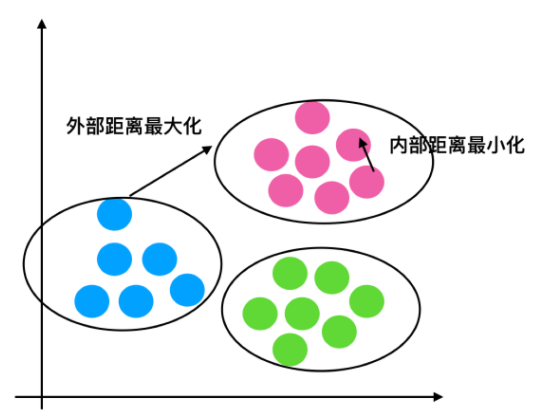6、K-means总结

• 特点分析：采用迭代式算法，直观易懂并且非常实用
• 缺点：容易收敛到局部最优解(多次聚类)

6、模型保存与加载

（1）APL

• import joblib

• 保存：joblib.dump(rf, ‘test.pkl’)

# 保存模型
joblib.dump(estimator,"my.pkl")

[外链图片转存失败,源站可能有防盗链机制,建议将图片保存下来直接上传(img-H2ipy9ct-1637326542975)(https://i.loli.net/2021/11/19/WFeOLAlRgrdxQjG.png)]

# 加载模型

Means(n_clusters=8,init=‘k-means++’)

• k-means聚类
• n_clusters:开始的聚类中心数量（也就是K值）
• init:初始化方法，默认为’k-means ++’
• labels_:默认标记的类型，可以和真实值比较（不是值比较）

4、对Instacart Market用户进行聚类

from sklearn.cluster import KMeans
import pandas as pd
import numpy as np
from sklearn.preprocessing import StandardScaler
from sklearn.metrics import silhouette_score#轮廓系数
def kmeans_demo():
"""
使用kmeans对用户种类进行预测
:return:
"""
# 导数据
cloumn_names = ['Sample code number', 'Clump Thickness', 'Uniformity of Cell Size', 'Uniformity of Cell Shape',
'Single Epithelial Cell Size', 'Bare Nuclei', 'Bland Chromatin', 'Normal Nucleoli', 'Mitoses',
'Class']

# 数据处理(缺失值处理）
# 替换为np.nan
data = data.replace(to_replace='?', value=np.nan)
# 删除nan所在的行
data.dropna(inplace=True)
# 查看是否有缺失值
# print(data.isnull().any())

data_new = data.iloc[:,:-1]
# 特征工程（无量纲）
transfer = StandardScaler()
data_new  = transfer.fit_transform(data_new)

# kmeans模型进行训练
estimator = KMeans(n_clusters=3)
estimator.fit(data_new)#只需要传入特征值
pre = estimator.predict(data_new)
print(pre[:300])

# 对模型训练结果进行评估--轮廓系数
score = silhouette_score(data_new,pre)
print("轮廓系数:\n",score)

return  None
if __name__=="__main__":
kmeans_demo()

5、轮廓系数

S C i = b i − a i m a x ( b i , a i ) SC_i= \frac{b_i-a_i}{max(b_i,a_i)}

注 ： 对 于 每 个 点 i 为 已 聚 类 数 据 中 的 样 本 ， b i 为 i 到 其 它 族 群 的 所 有 样 本 的 距 离 最 小 值 ， a i 为 i 到 本 身 簇 的 距 离 平 均 值 。 最 终 计 算 出 所 有 的 样 本 点 的 轮 廓 系 数 平 均 值 注：对于每个点i 为已聚类数据中的样本 ，b_i 为i 到其它族群的所有样本的距离最小值，a_i 为i 到本身簇的距离平均值。最终计算出所有的样本点的轮廓系数平均值

如 果 b i > > a i : 趋 近 于 1 效 果 越 好 ， b i < < a i : 趋 近 于 − 1 ， 效 果 不 好 。 轮 廓 系 数 的 值 是 介 于 [ − 1 , 1 ] ， 越 趋 近 于 1 代 表 内 聚 度 和 分 离 度 都 相 对 较 优 。 如果b_i>>a_i:趋近于1效果越好， b_i<<a_i:趋近于-1，效果不好。轮廓系数的值是介于 [-1,1] ，越趋近于1代表内聚度和分离度都相对较优。

[外链图片转存中…(img-M80QbmPp-1637326542973)]

6、K-means总结

• 特点分析：采用迭代式算法，直观易懂并且非常实用
• 缺点：容易收敛到局部最优解(多次聚类)

6、模型保存与加载

（1）APL

• import joblib

• 保存：joblib.dump(rf, ‘test.pkl’)

# 保存模型
joblib.dump(estimator,"my.pkl")

[外链图片转存中…(img-H2ipy9ct-1637326542975)]

# 加载模型

展开全文python 机器学习
• 我们继续分别使用最小化函数fminunc()和自实现的梯度下降来进行机器学习训练。本篇的重点在于理解如何从单变量的统计回归模型推广到多变量模型中去，一旦理解了这一点，我们就可以将其推广到任意维度。 经过训练，...机器学习 逻辑回归
• 机器学习是一门多领域交叉学科，涉及概率论、统计学、逼近论、凸分析、算法复杂度理论等多门学科。专门研究计算机怎样模拟或实现人类的学习行为，以获取新的知识或技能，重新组织已有的知识结构使之不断改善自身的...机器学习 人工智能 算法 统计模型
• 恶意URL检测的方法很多，这里介绍通过机器学习分析URL文本分词词频来检测恶意URL。...具体实现过程包括数据载入–>数据处理（分词、向量化处理）–>模型训练–>模型保存–>模型应用 项目组织结构如下：机器学习 python 网络安全
•机器学习 人工智能 深度学习
• 代码实现 5.1 线性回归梯度下降法 二、逻辑回归 1.逻辑回归的介绍 1.1 假设陈述 1.2 决策界限 1.3 代价函数 1.3.1 代价函数介绍 1.3.2 简化代价函数 1.3.3 梯度下降求解最优Cost 2.逻辑回归模型的优劣势 3.高级优化 ...机器学习 算法 python
• 具体准备工作： 2019 年 2 月--2019 年 4 月：笔记整理初级阶段，视频 100% 复现 2019 年 4 月--2019 年 6 月：网站搭建，对笔记内容及排版迭代优化 2019 年 5 月--2019 年 6 月：组队学习《李宏毅机器学习》并对...人工智能 编程语言 机器学习 深度学习 大数据
• 机器学习的7个步骤

千次阅读 2021-05-09 00:46:26
点击上方“小白学视觉”，选择加"星标"或“置顶”重磅干货，第一时间送达 机器学习是技术爱好者中高度关注的领域。作为人工智能（AI）的一个分支，它基本上是一种算法或模型，可...大数据 算法 编程语言 python 计算机视觉机器学习 深度学习
• 对于给定的训练集，首先基于特征条件独立假设学习输入输出的联合概率分布（朴素贝叶斯法这种通过学习得到模型的机制，显然属于生成模型）；然后基于此模型，对给定的输入 x，利用贝叶斯定理求出后验概率最大的输出 y...机器学习 分类 人工智能
• 机器学习15_线性回归算法详解 （2021.05.30） 一. 基础知识 什么是回归问题？回归问题的本质又是什么？ 回归问题其实就是目标值是连续性的值，而分类问题的目标值则是离散型的值。回归处理的问题为预测，其本质都...机器学习 算法 python
• 主要代码参考于此，感谢b站大学 主要代码参考于此，感谢GitHub老师 本篇主要记录一下几种常用的降维算法 数据是darmanis数据集，包括466个细胞2000个高表达量基因，分为九种类型的细胞集群。数据部分截图： 其中行为...机器学习 聚类 pythonpytorch python 机器学习
• 以下是具体实现代码 """ import torch import torch as t from matplotlib import pyplot as plt t.manual_seed(100) dtype = t.float # 生成x坐标数据，x为tensor，需要把x的形状转换为100*1 x...机器学习 人工智能
• 逻辑回归案例二：鸢尾花数据分类，决策边界绘制逐步代码讲解1 数据加载2 数据EDA3 模型创建及应用3.1 数据切分3.2 创建模型与分类3.3 决策边界绘制3.3.1 二分类决策边界绘制3.3.2 多分类决策边界绘制3.3.3 三维决策...机器学习 逻辑回归 分类 决策边界
• 机器学习之监督学习-分类模型K近邻(KNN)算法实现 监督学习中常用的分类分类模型有k近邻，逻辑斯谛回归，决策树。今天我们主要学习，K近邻模型。 最简单，最初级的分类器就是将全部的训练数据所对应的类别都记录下来...机器学习 分类 算法
• 课题：特征工程理论及代码实现 日期：2019.9.21 作者：小知同学 描述：本篇比较详细的介绍了特征工程的理论以及代码实现，涵盖数据预处理、特征选择、特征构造、特征降维、 类别不平衡处理等内容，不仅介绍了相关...python 机器学习 特征工程 特征选择 异常值
• 目录前言PMML概念使用JPMML的操作步骤训练模型——jpmml-sklearn相关项目仓库安装Python库生成pmml模型三步曲第一步——创建模型第二步——训练模型第三步——保存模型回归任务演示代码部署模型——jpmml-...flink 机器学习 kafka flume
• 说明：这是一个机器学习实战项目（附带数据+代码），如需数据+完整代码可以直接到文章最后获取。 1.问题定义 在日常银行、电商等公司中，随着时间的推移，都会积累一些客户的数据。在当前的大数据时代、人工...聚类算法 聚类 机器学习 python 项目实战
• Python机器学习房价预测 (斯坦福大学机器学习课程) 机器学习的步骤简单的分为以下三步 步骤①数据获取与处理 步骤②选择与训练模型 步骤③评估与显示 一. 对于文章涉及知识的学习 （1）数据处理——数据标准化 1.为...机器学习 python 人工智能 算法
• 使用机器学习处理信号，我以语音信号为例。常见的声学信号特征有： 这些是声学信号中，常见的手工特征。针对特定的信号，会有一些常用的特征。一些通用的方法有：小波分解，信号分解（提取信号不同频率的特质）、...机器学习 信号处理
• 【导读】：全面介绍机器学习发展的历史，从感知机、神经网络、决策树、SVM、Adaboost到随机森林、Deep Learning。 自科学技术和人工智能最初发展开始，科学家Blaise Pascal和Von Leibniz就思考着如何制造一台像...人工智能 机器学习 神经网络 python
• 文章目录一、什么是机器学习二、机器学习的流程（一）数据收集（二）数据预处理（三）特征工程（四）模型构建和训练三、机器学习该如何学（一）AI时代首选Python（二）PyCharm可视化编辑器和Anaconda大礼包1、...机器学习 人工智能 自然语言处理
• 韩智 博雯 发自 凹非寺量子位 报道 | 公众号 QbitAI不写代码也能搞机器学习？！这是亚马逊云科技在最近亚马逊云科技 re:Invent 大会上公布的新工具，用于实现代码可视化机器...大数据 编程语言 机器学习 人工智能 数据分析
• 它的发生正是很多机器学习算法工作的原理。它会去寻找最能区分两个类别的、最明显的特征。这里，A品牌与B品牌最明显的区别是A都是朝向左侧，B是朝向右侧。 你的神经网络会与你喂给它的数据质量一样好或坏。 我们...机器学习 人工智能
• airflow架构3.airflow核心模块3.1 模块3.2 Operators模块4.airflow安装与使用4.1 安装airflow4.2 修改默认路径4.3 修改默认数据库4.4 初始化...用户4.6 启动web服务4.7 启动定时任务4.8 编写airflow自动化代码模版...python 机器学习 sklearn
• 机器学习-数据预处理 1.概述 1.1数据挖掘的五大流程 获取数据 数据预处理：从数据中检测，纠正或删除损坏，不准确或不适用于模型的记录的过程 可能面对的问题有：数据类型不同，比如有的是文字，有的是数字，...机器学习...